# ML Aggarwal Solutions for Class 8 Maths Chapter 4 Cubes and Cube Roots

ML Aggarwal Solutions for Class 8 Maths Chapter 4 Cubes and Cube Roots are present here to help students prepare for their exams and score well. This chapter mainly deals with problems based on finding cubes and cube roots of numbers and expressions. To aid students in developing their problem-solving abilities, the solutions are created in a step by step format by an expert faculty team at BYJU’S, having vast academic experience. Practising these solutions on a regular basis builds confidence among students to crack the difficult problems during exams. ML Aggarwal Solutions is one of the best reference materials one can opt for to prepare for various exams, which yields excellent results. The solutions PDF is readily available for students and can be downloaded from the link given below easily.

Chapter 4 Cubes and Cube Roots contains two exercises and Check your progress questions for which the ML Aggarwal Class 8 Solutions present on this page provide precise solutions. Moreover, these solutions are in accordance with the latest ICSE guidelines to help students secure maximum marks possible.

## Download the PDF of ML Aggarwal Solutions for Class 8 Maths Chapter 4 Cubes and Cube Roots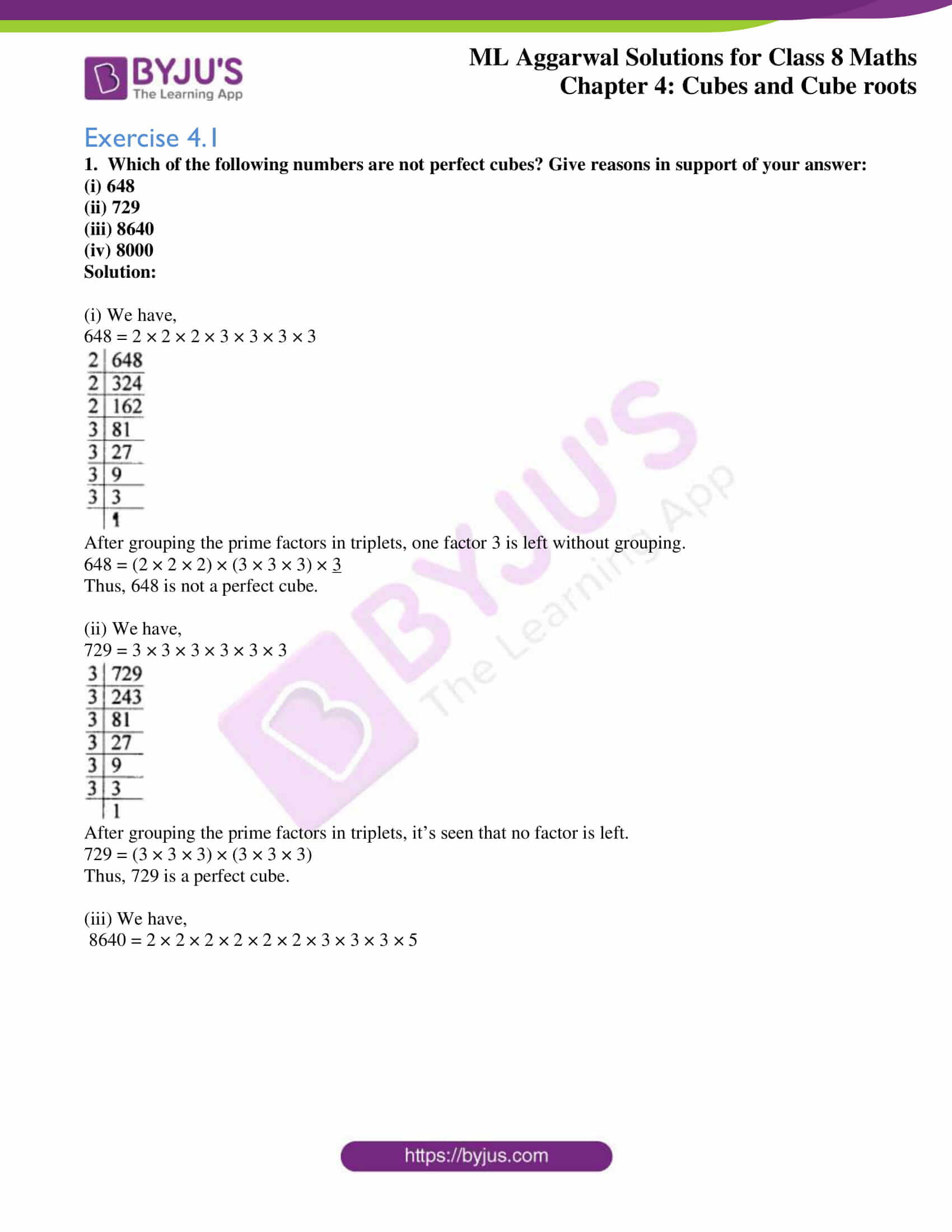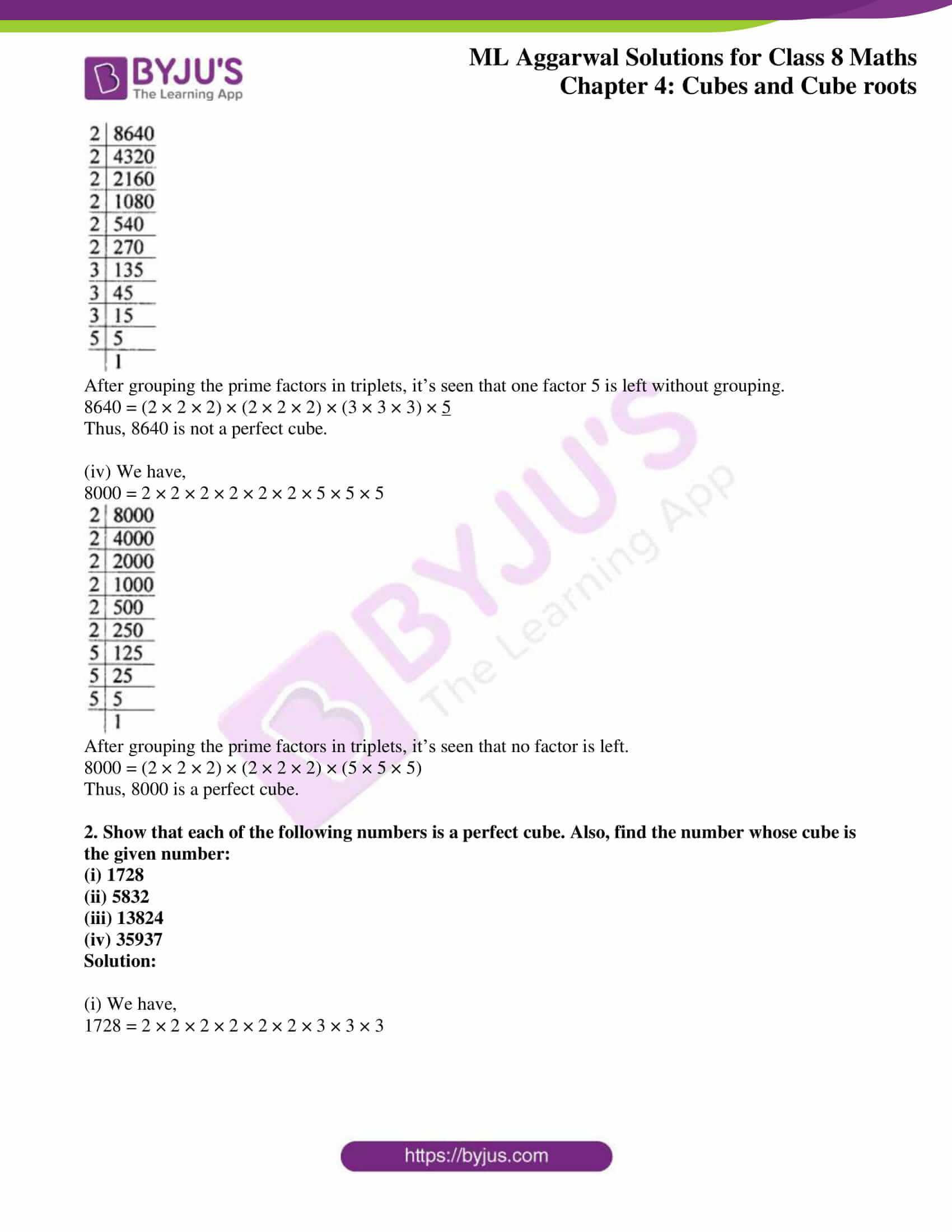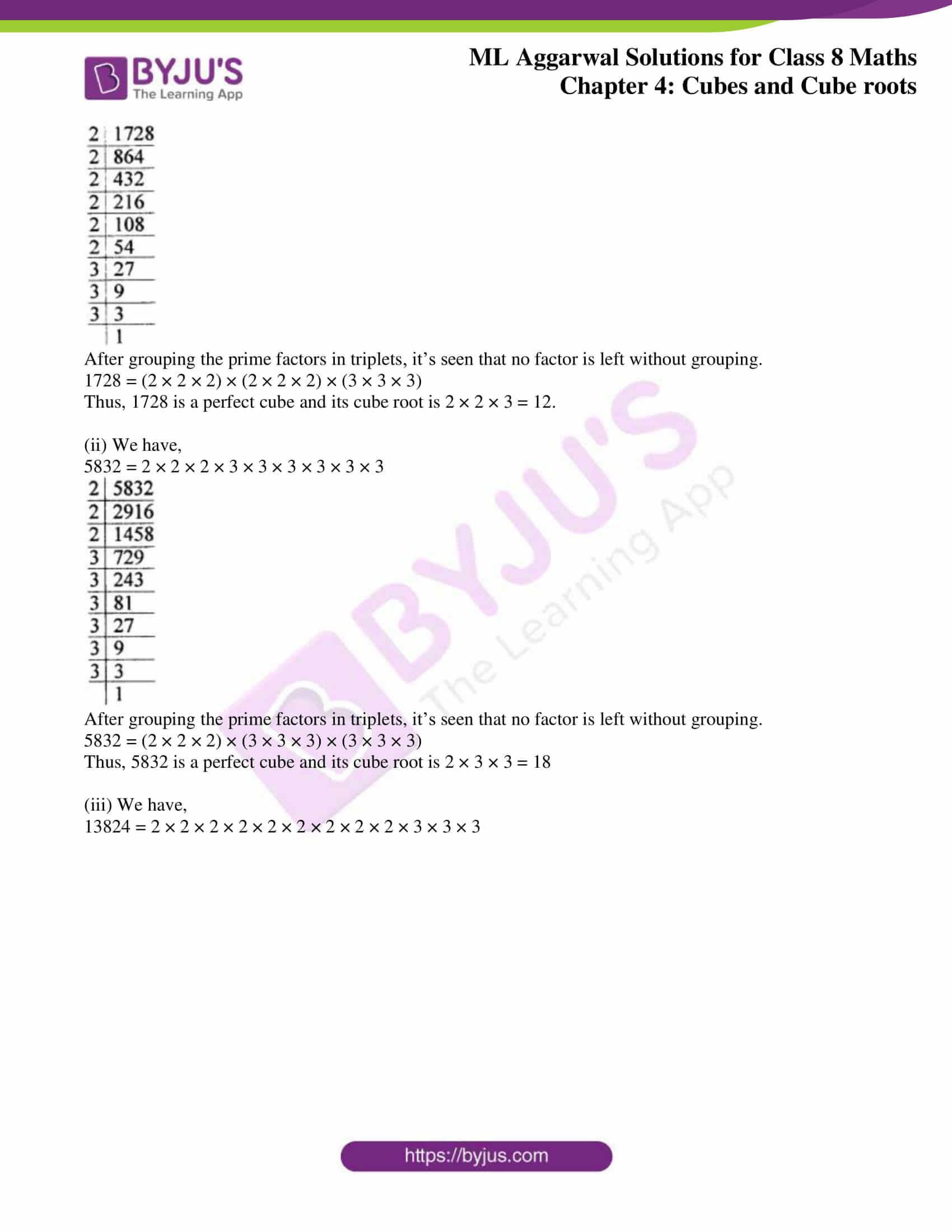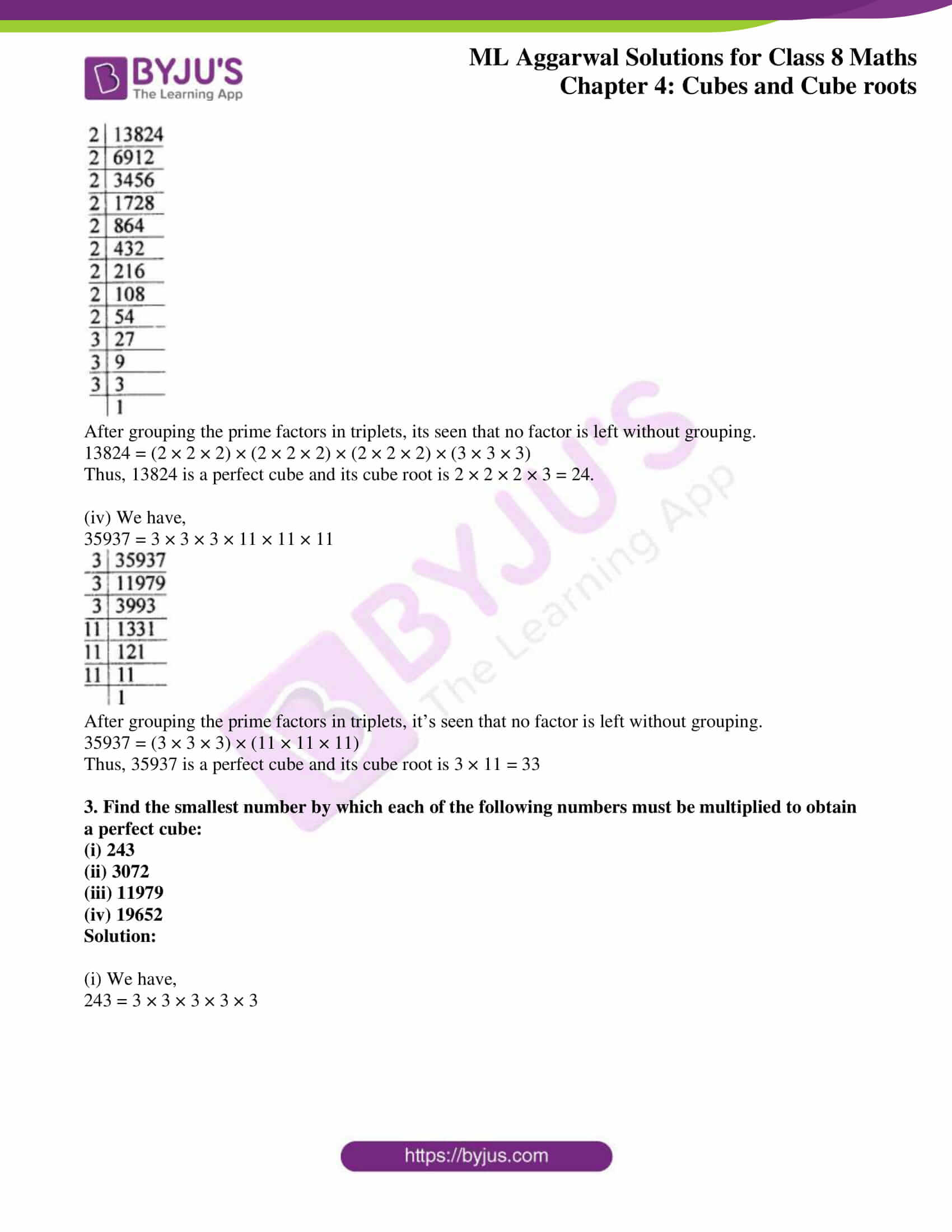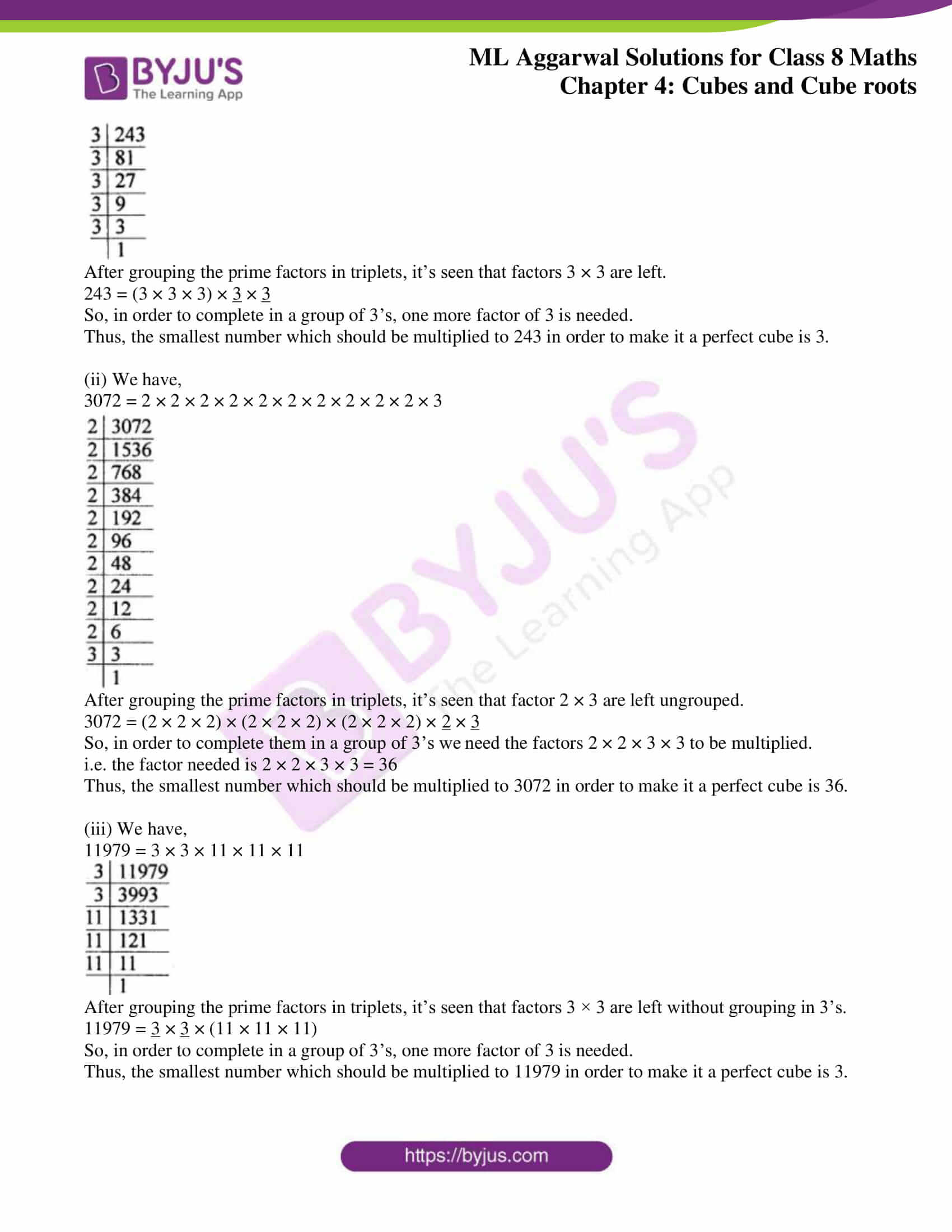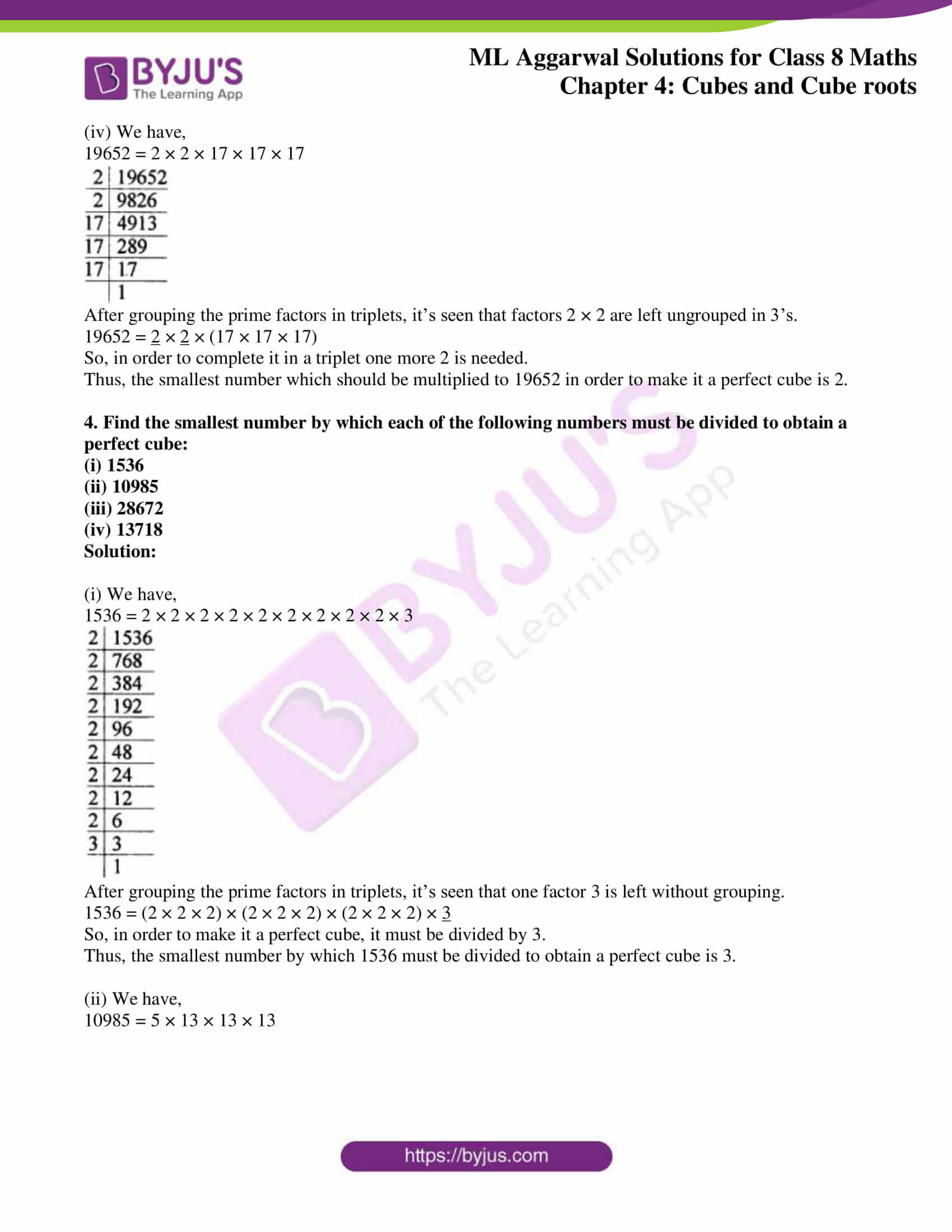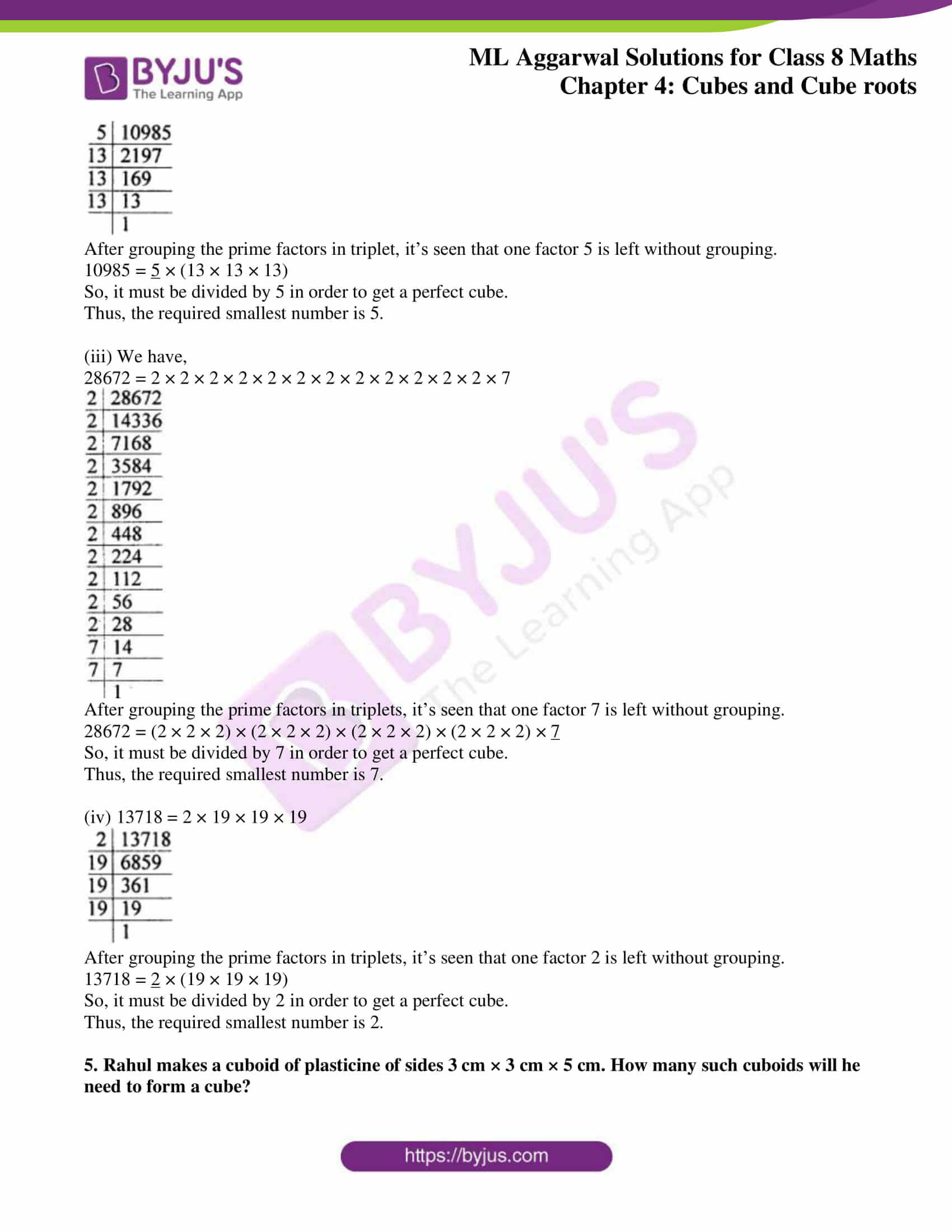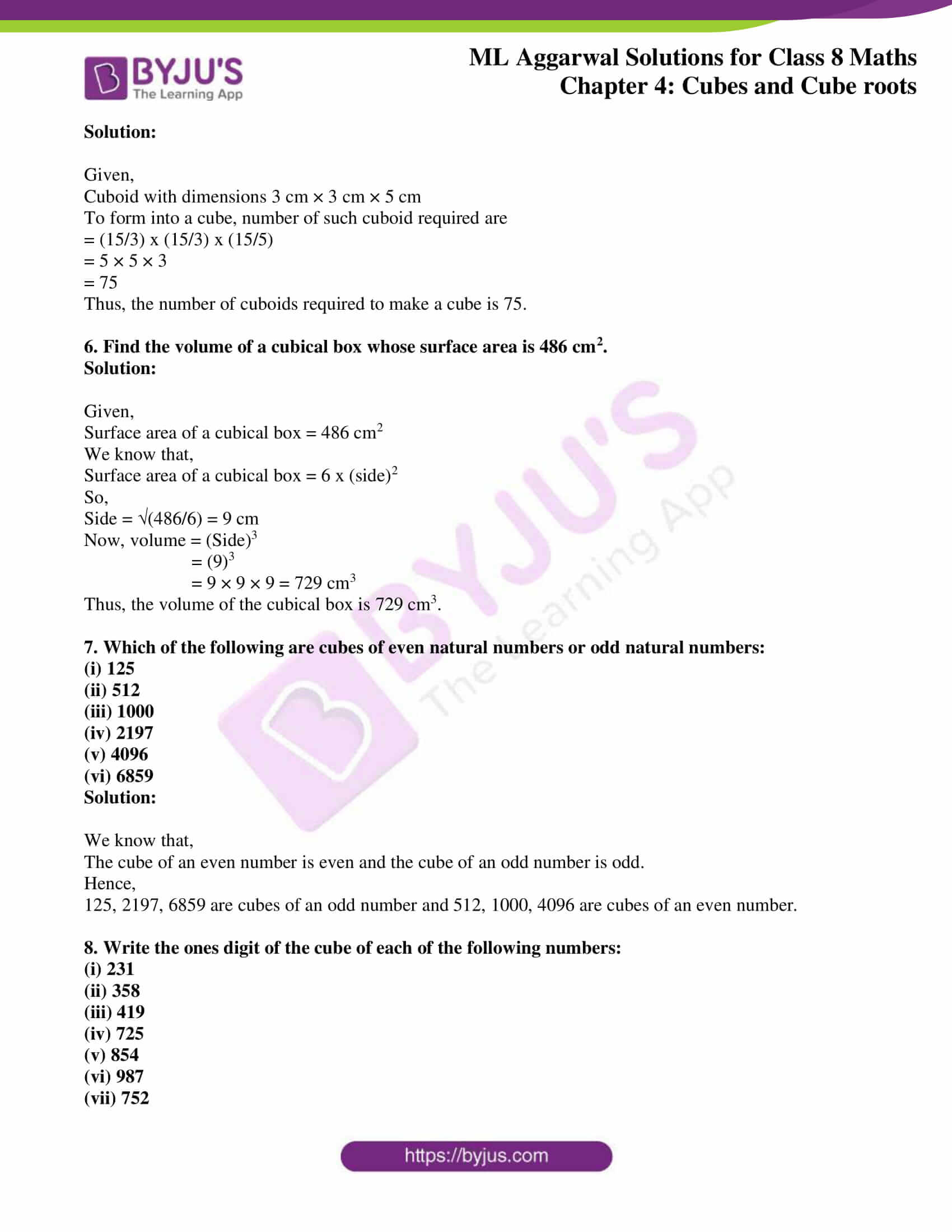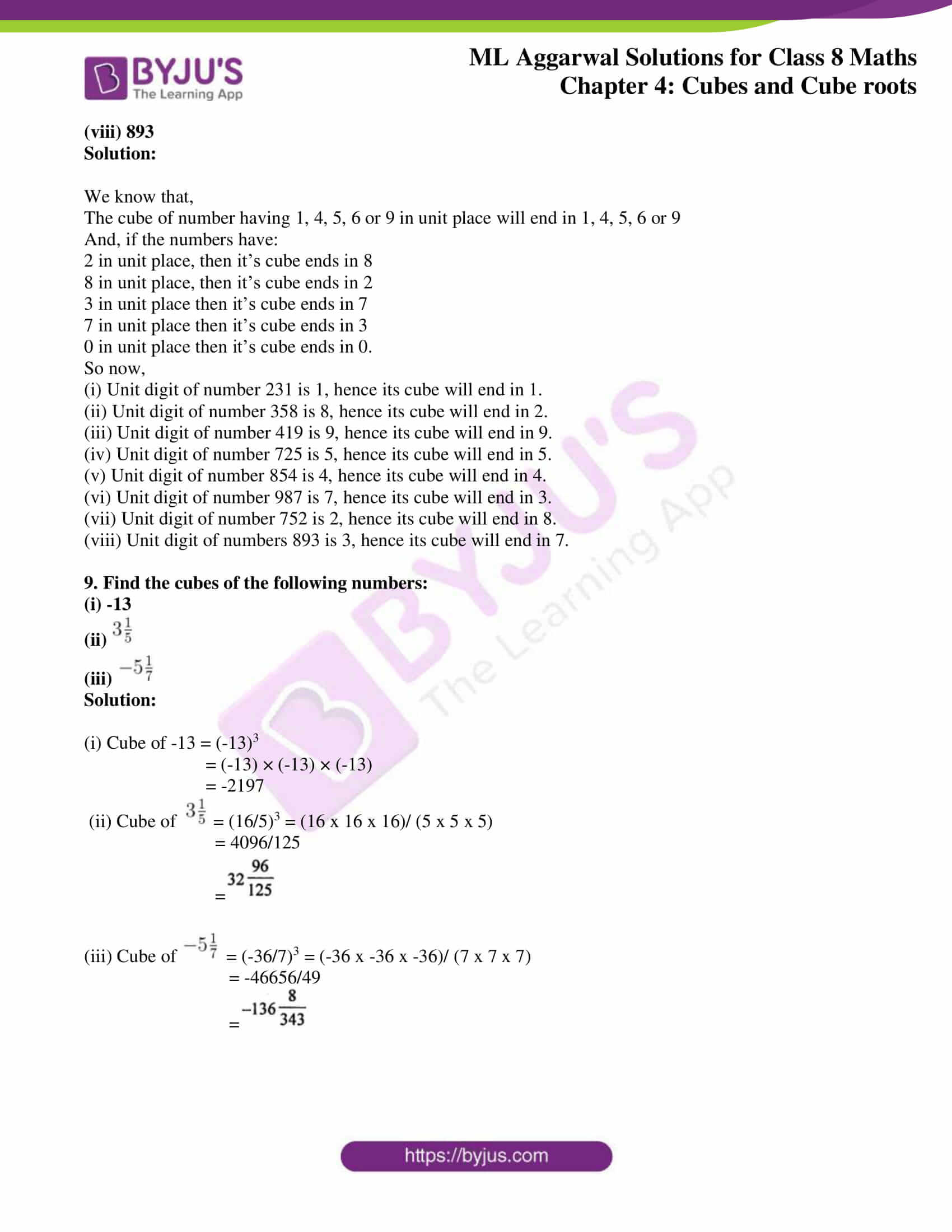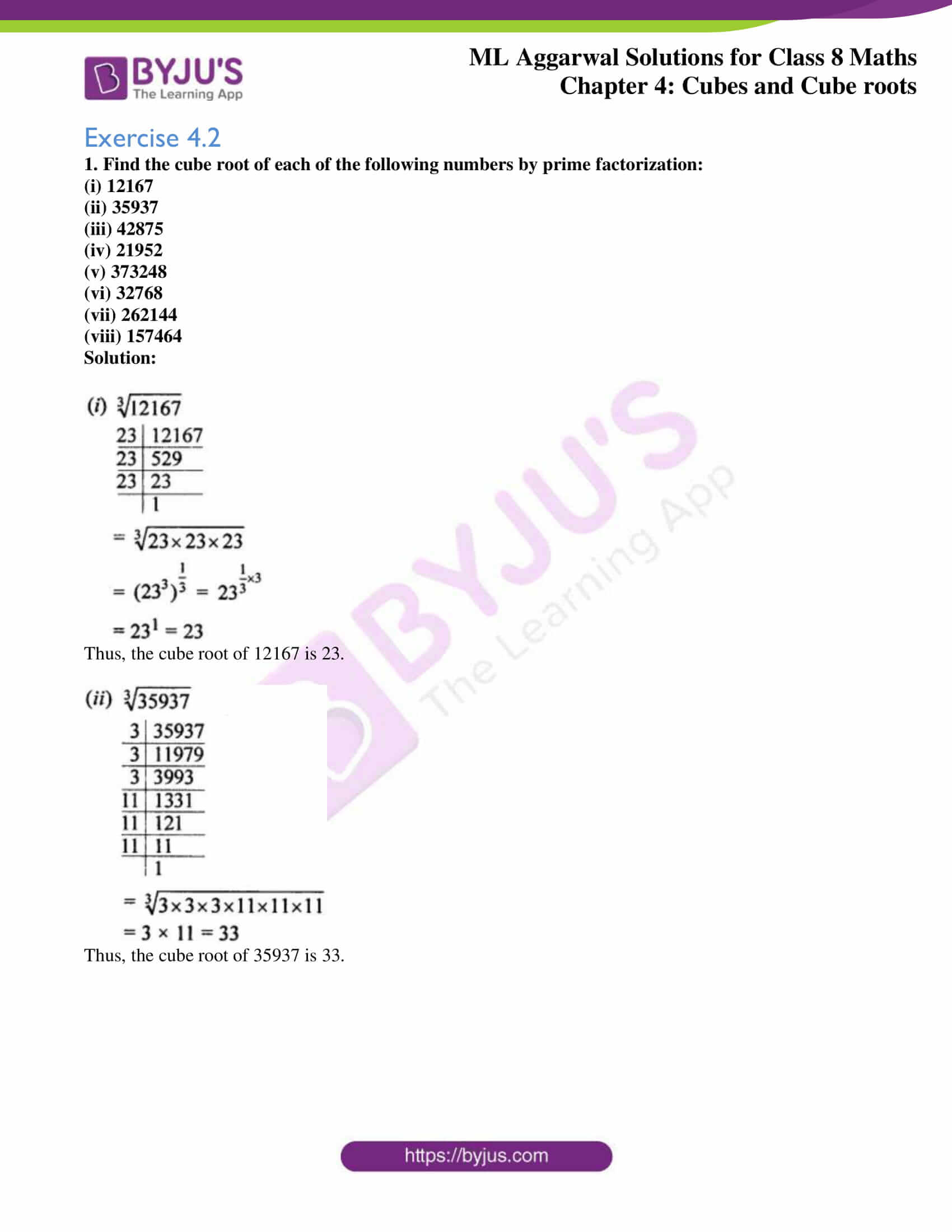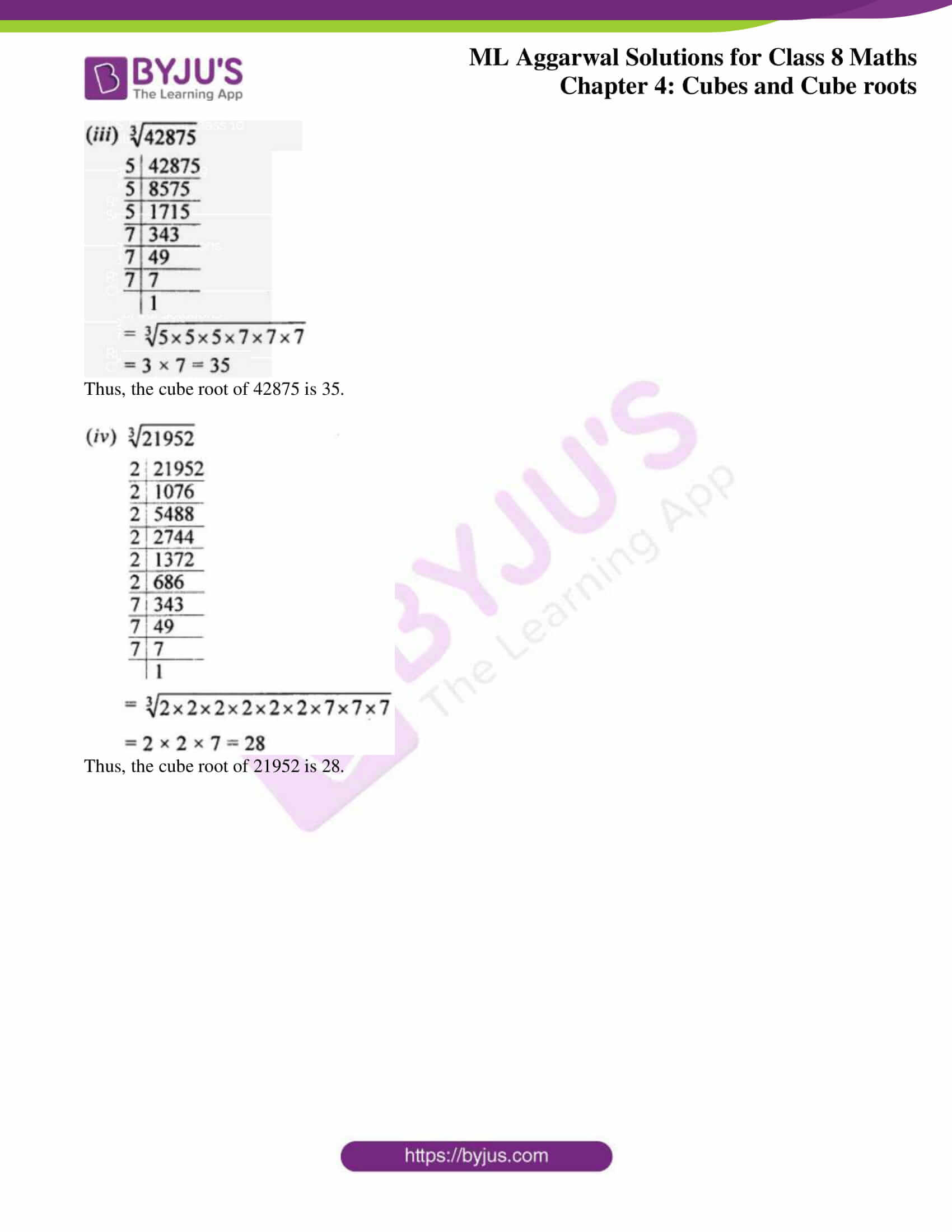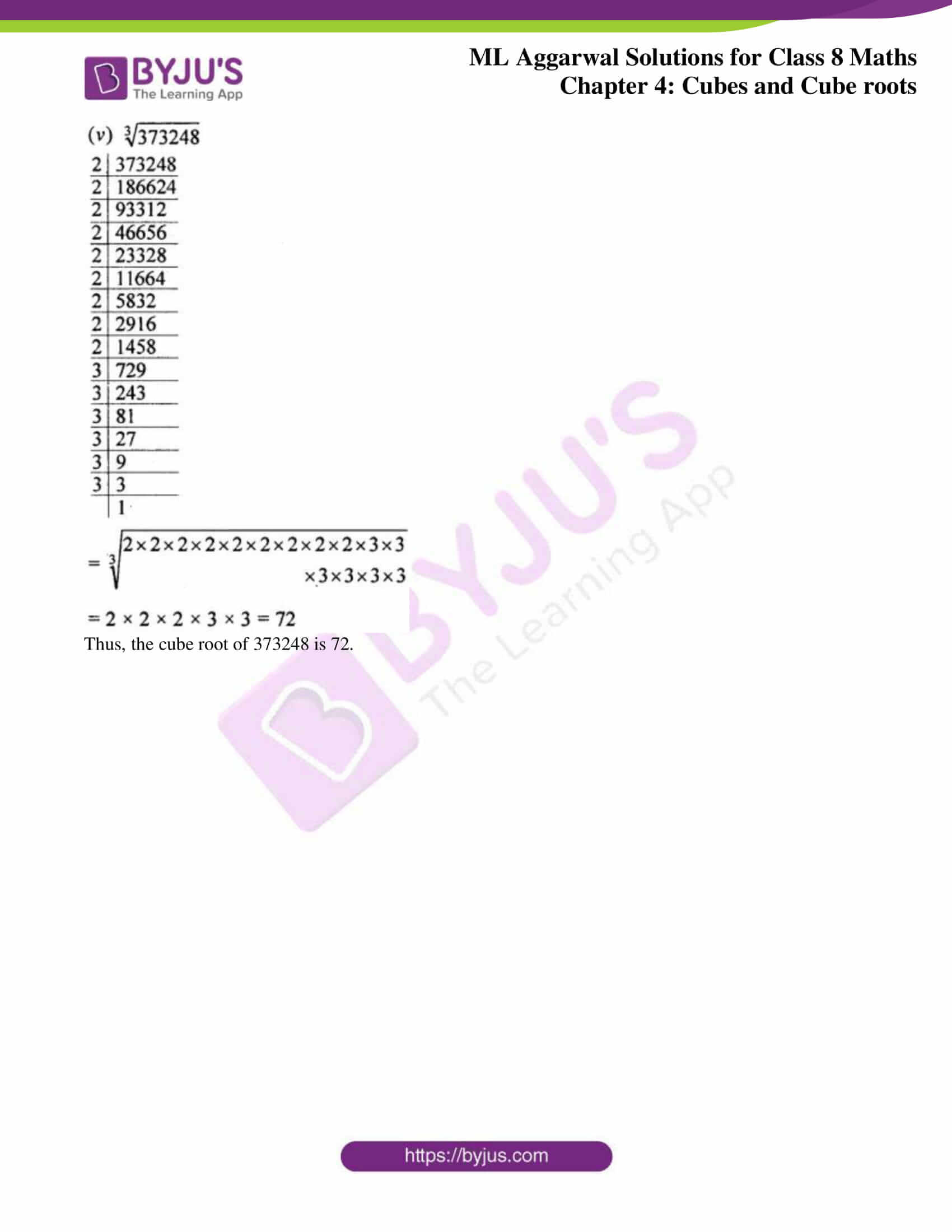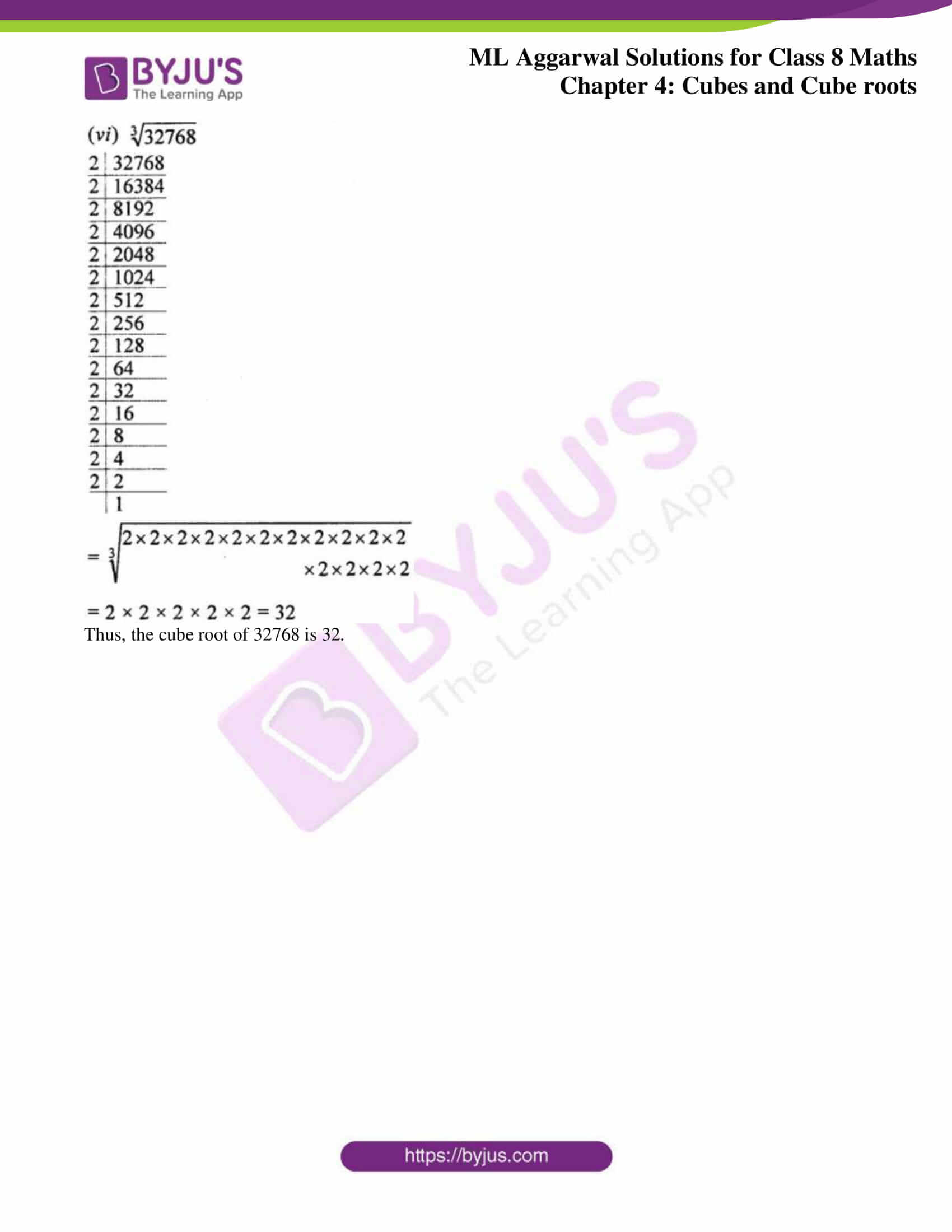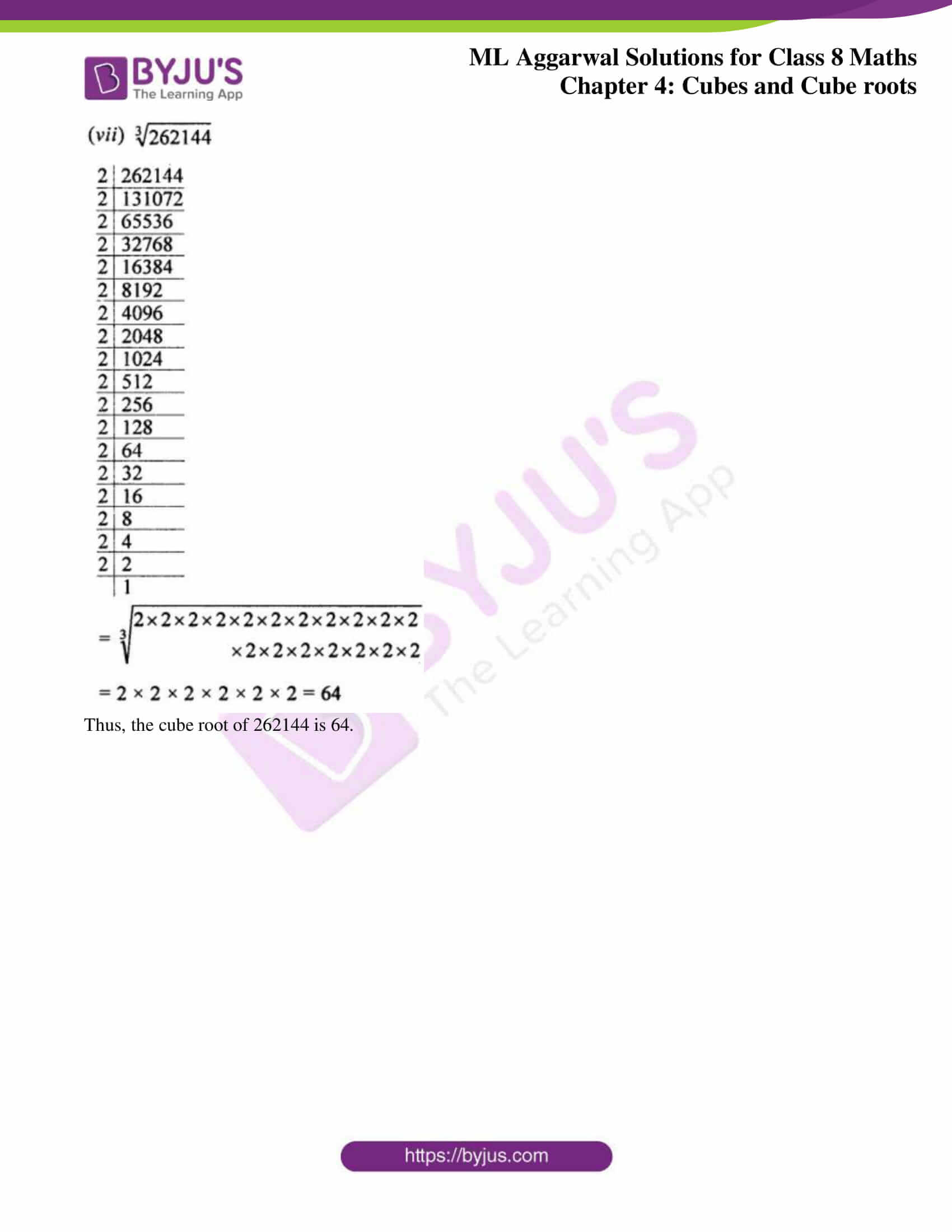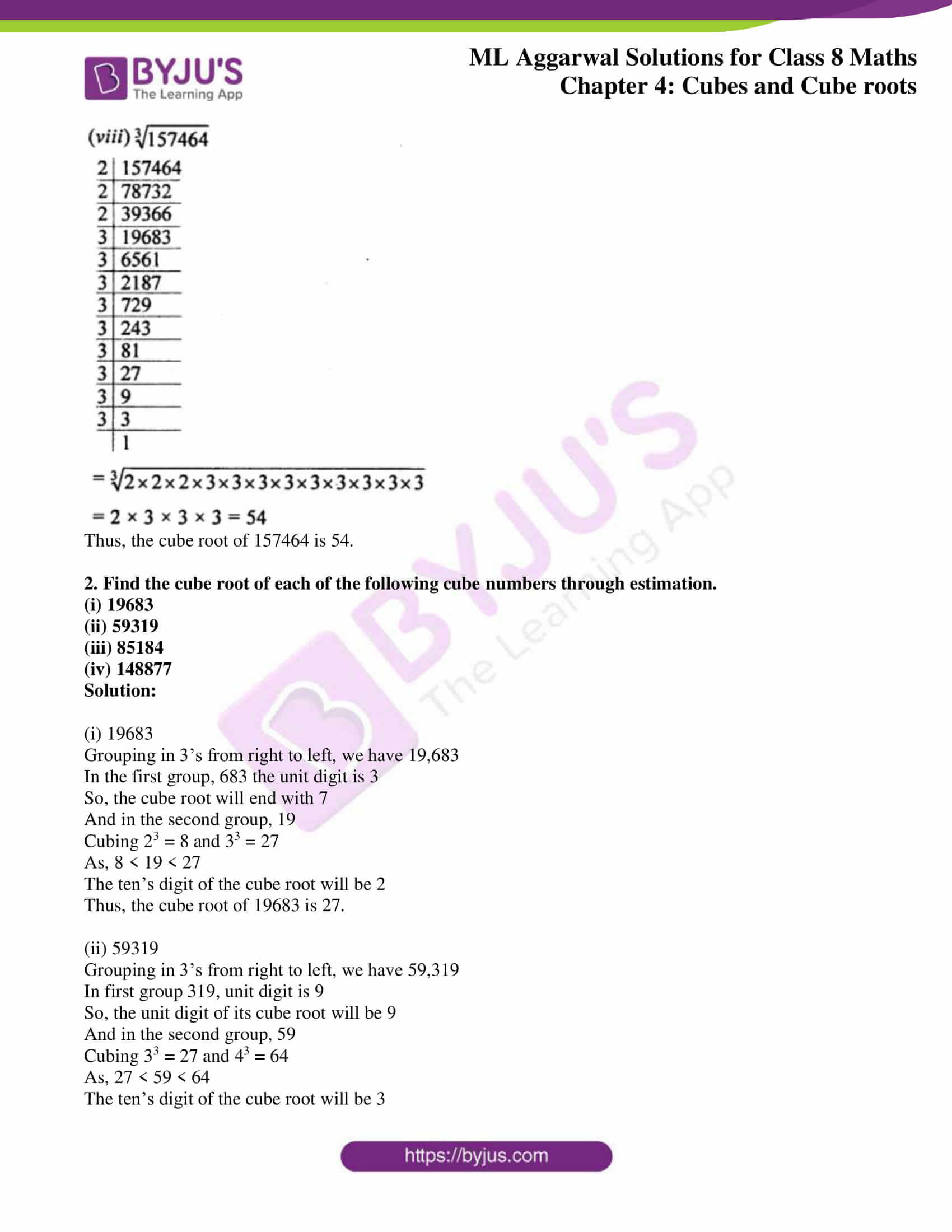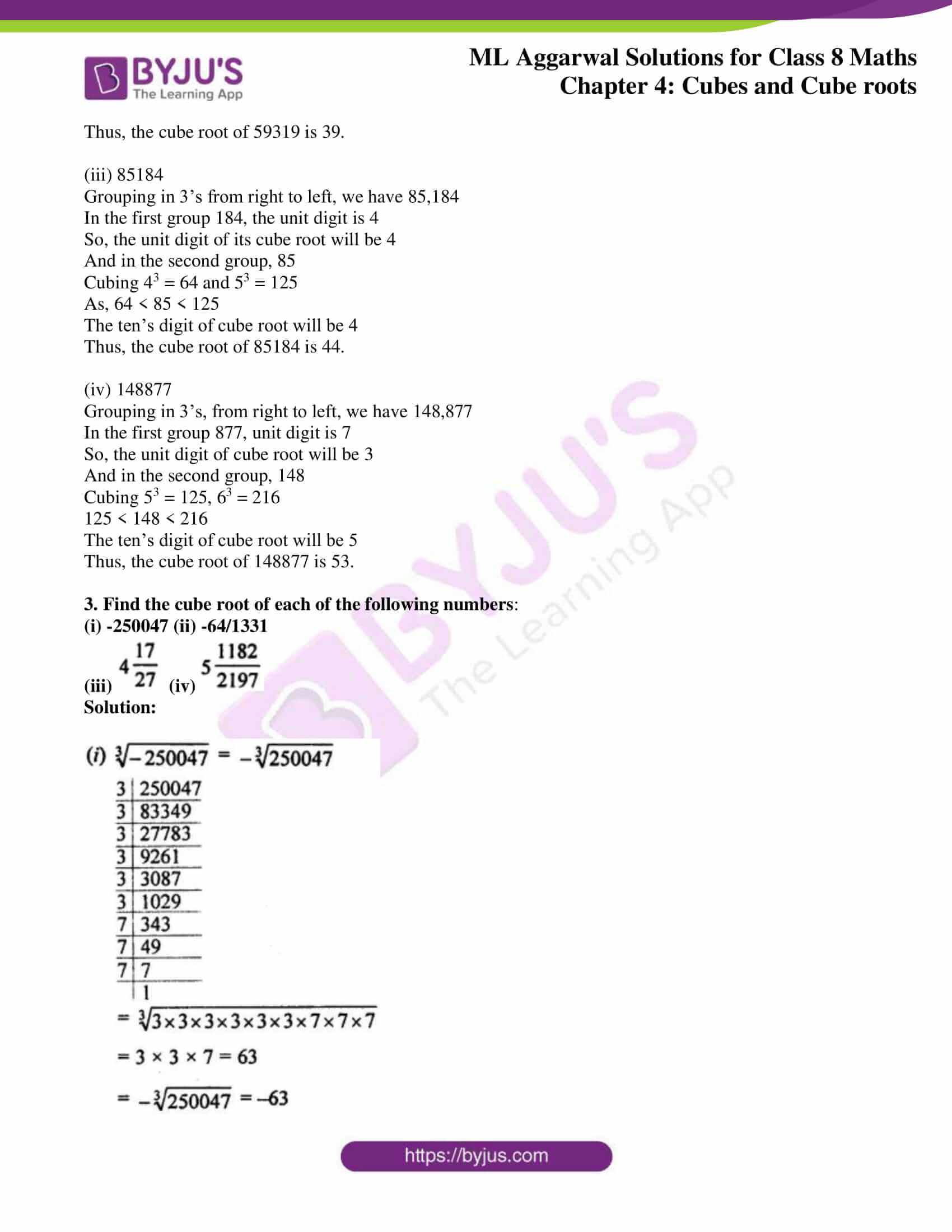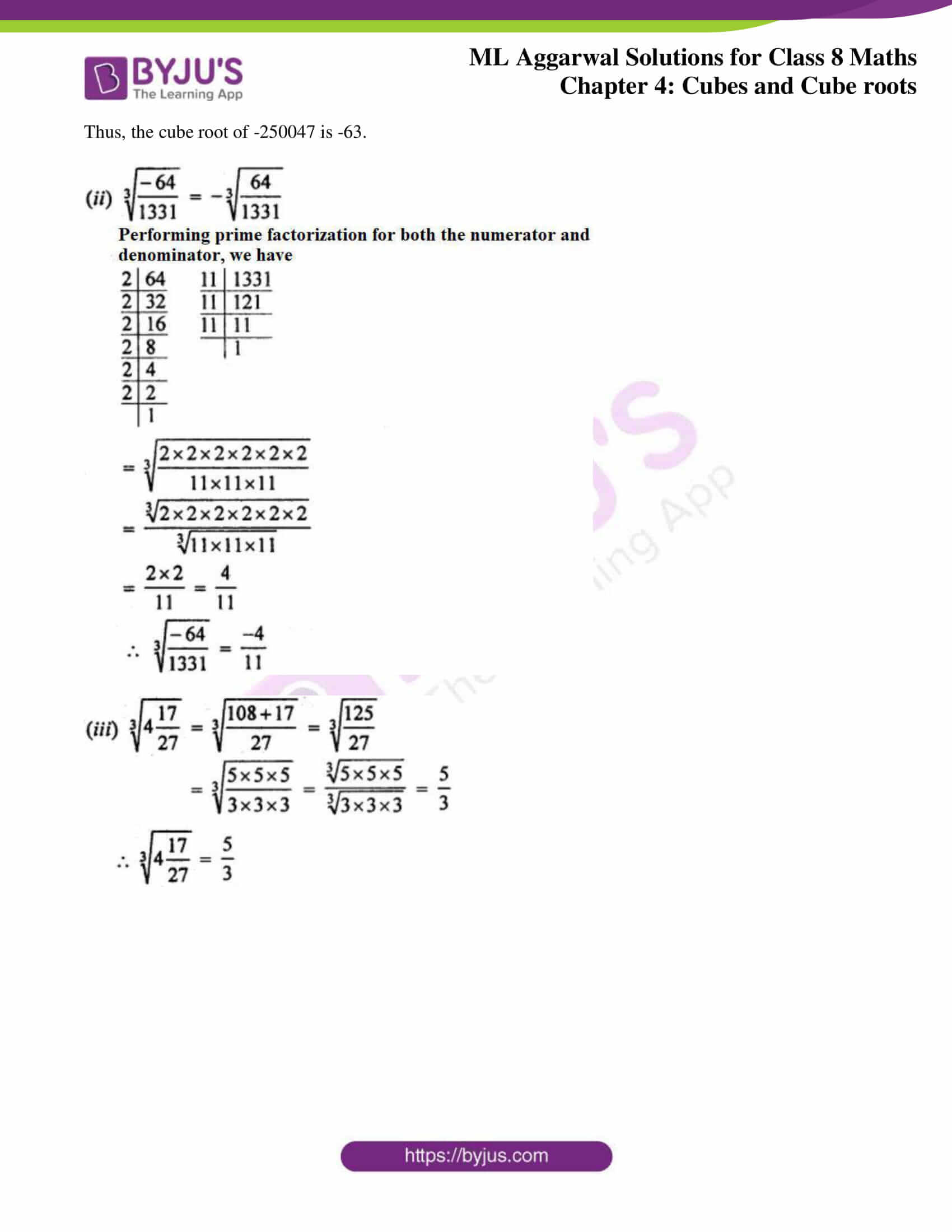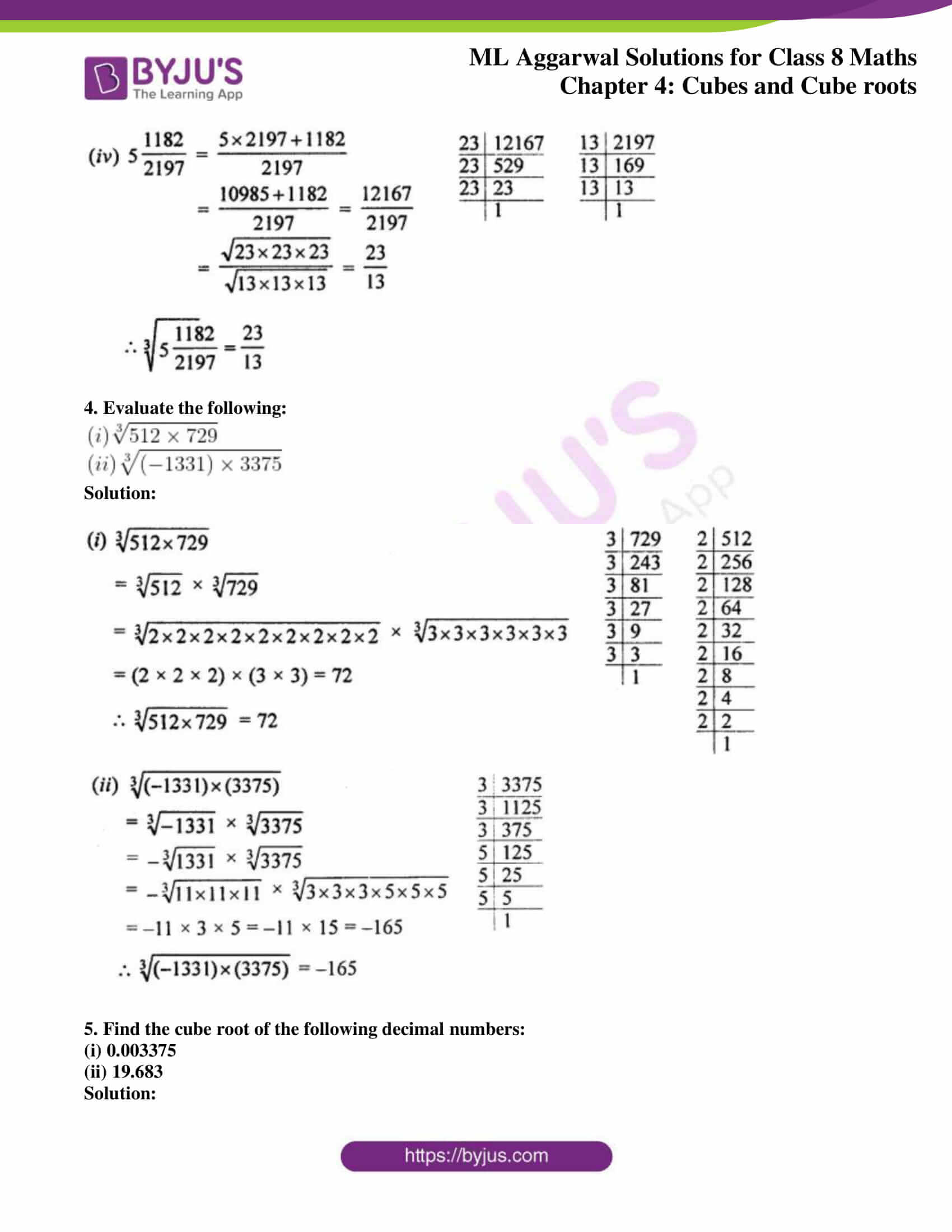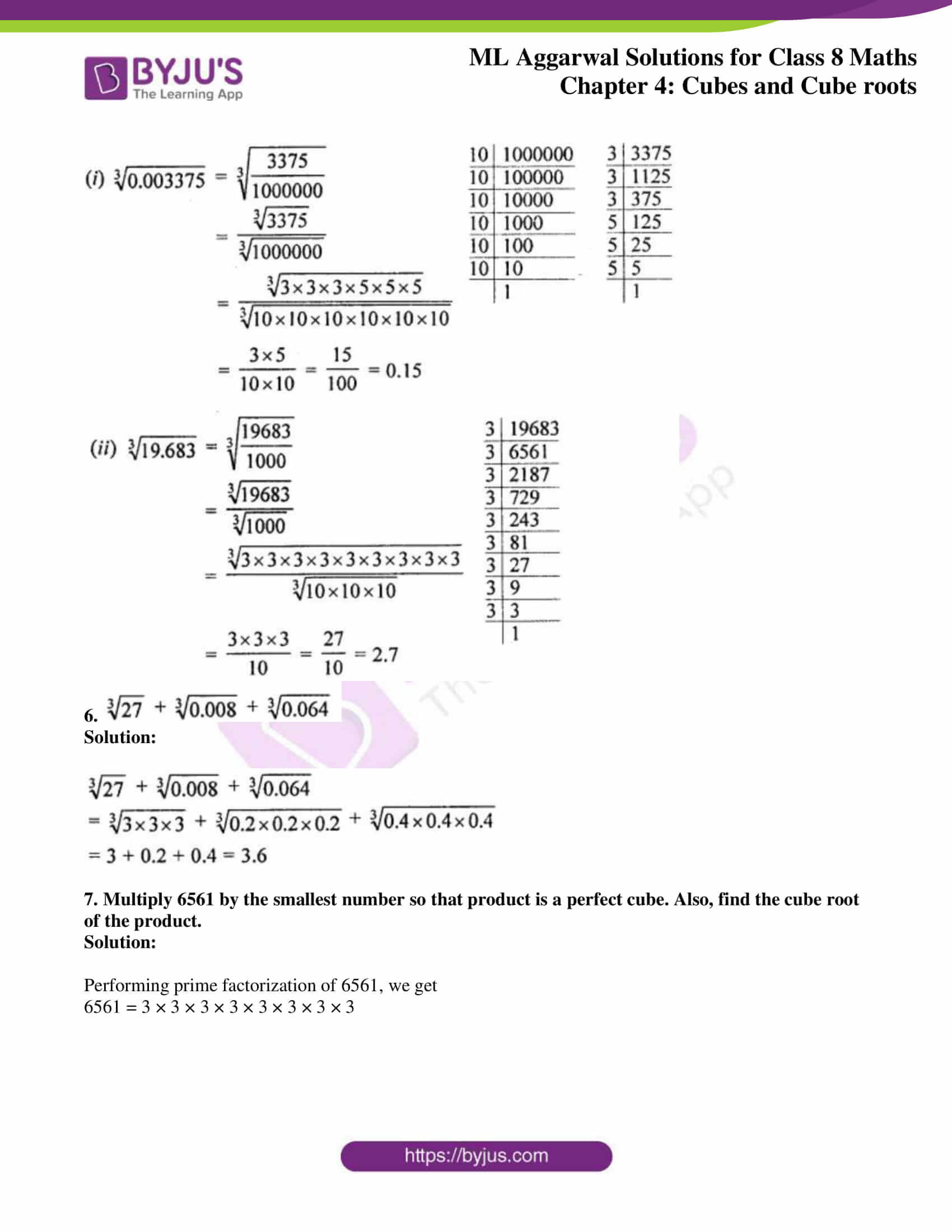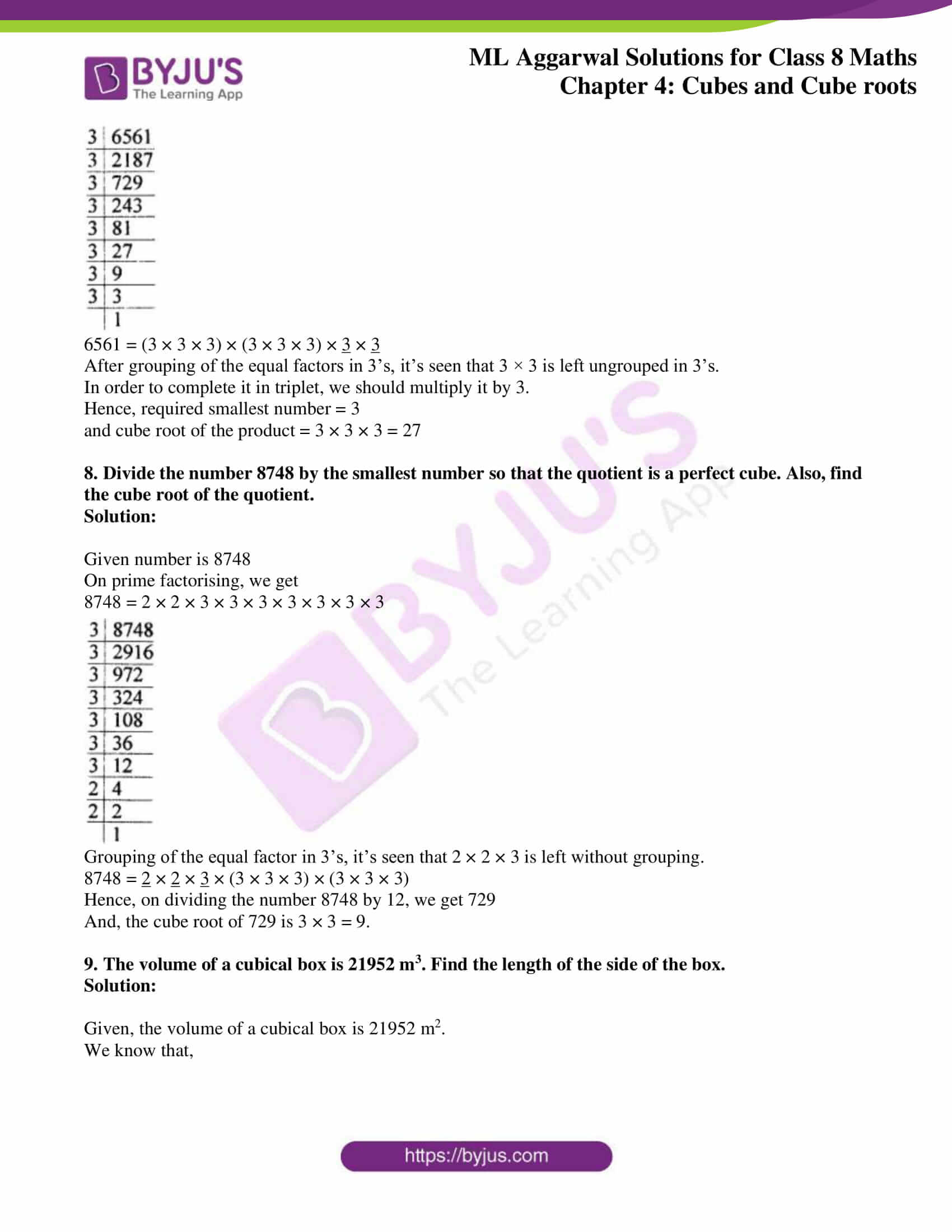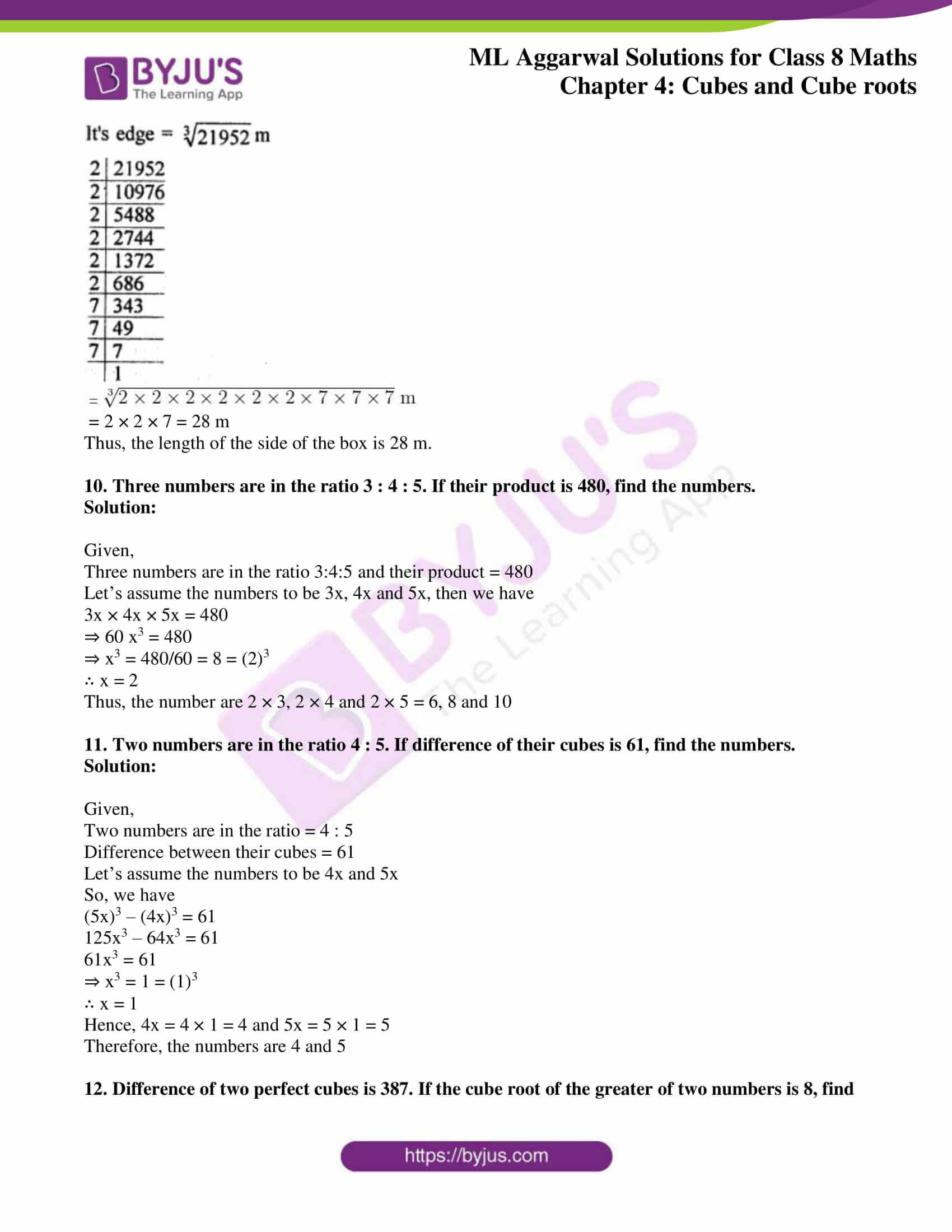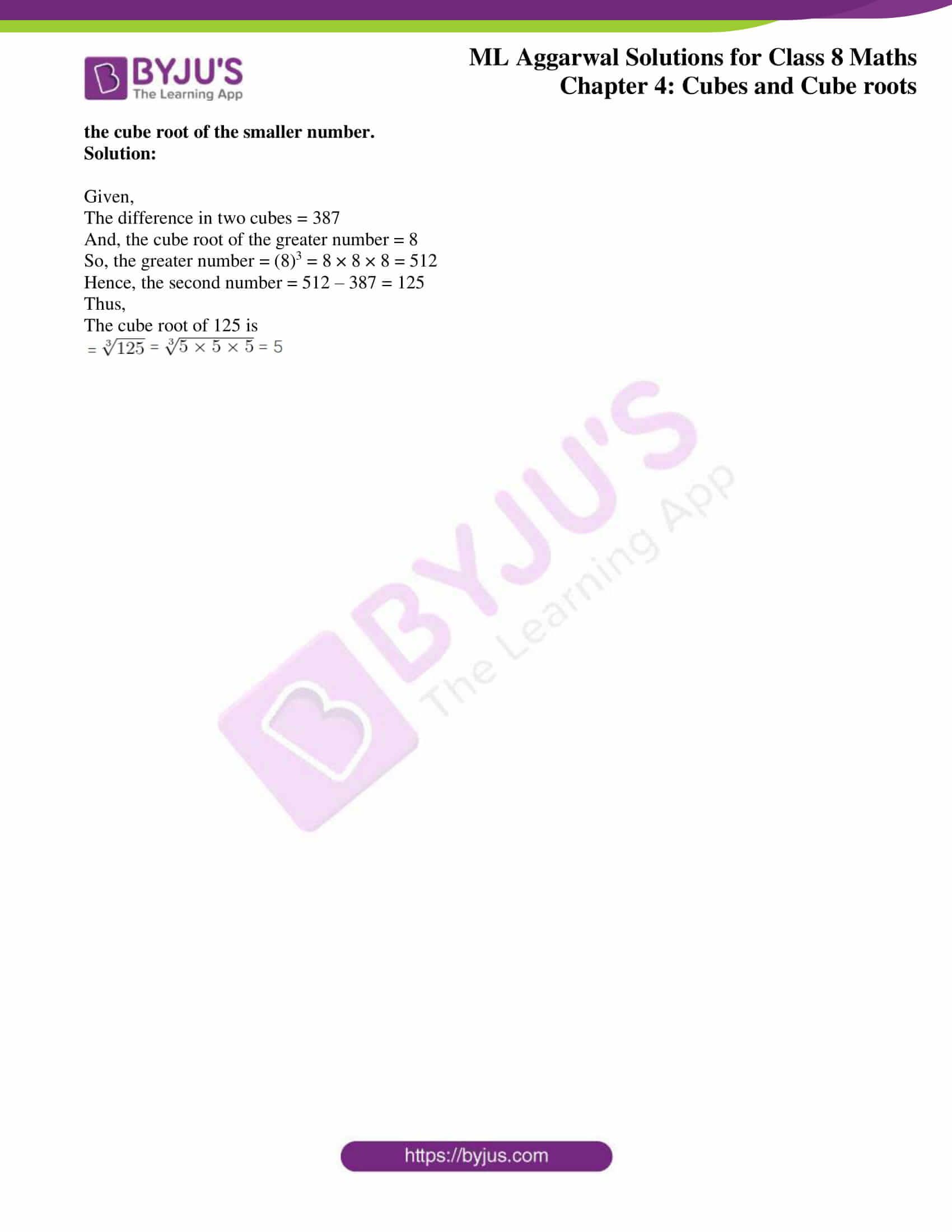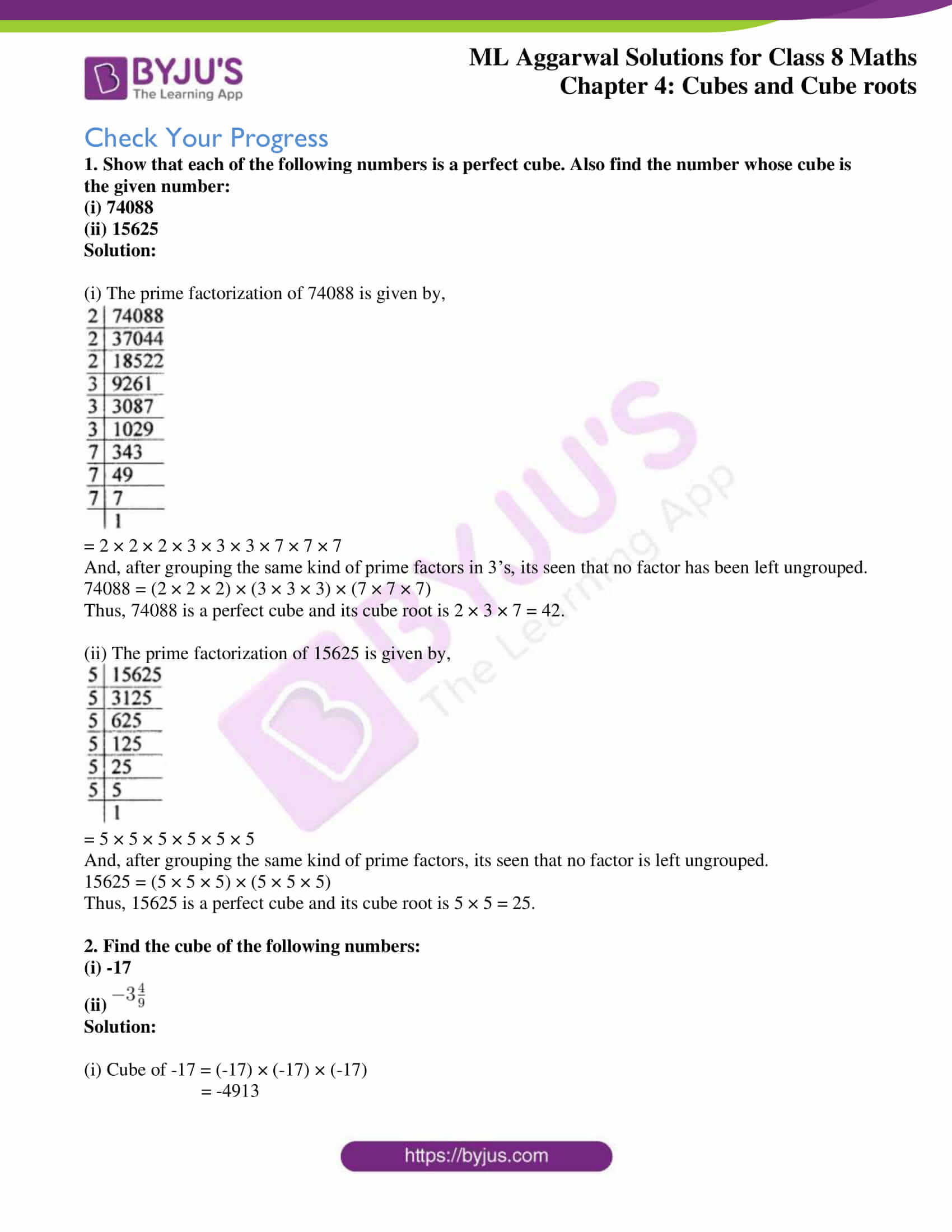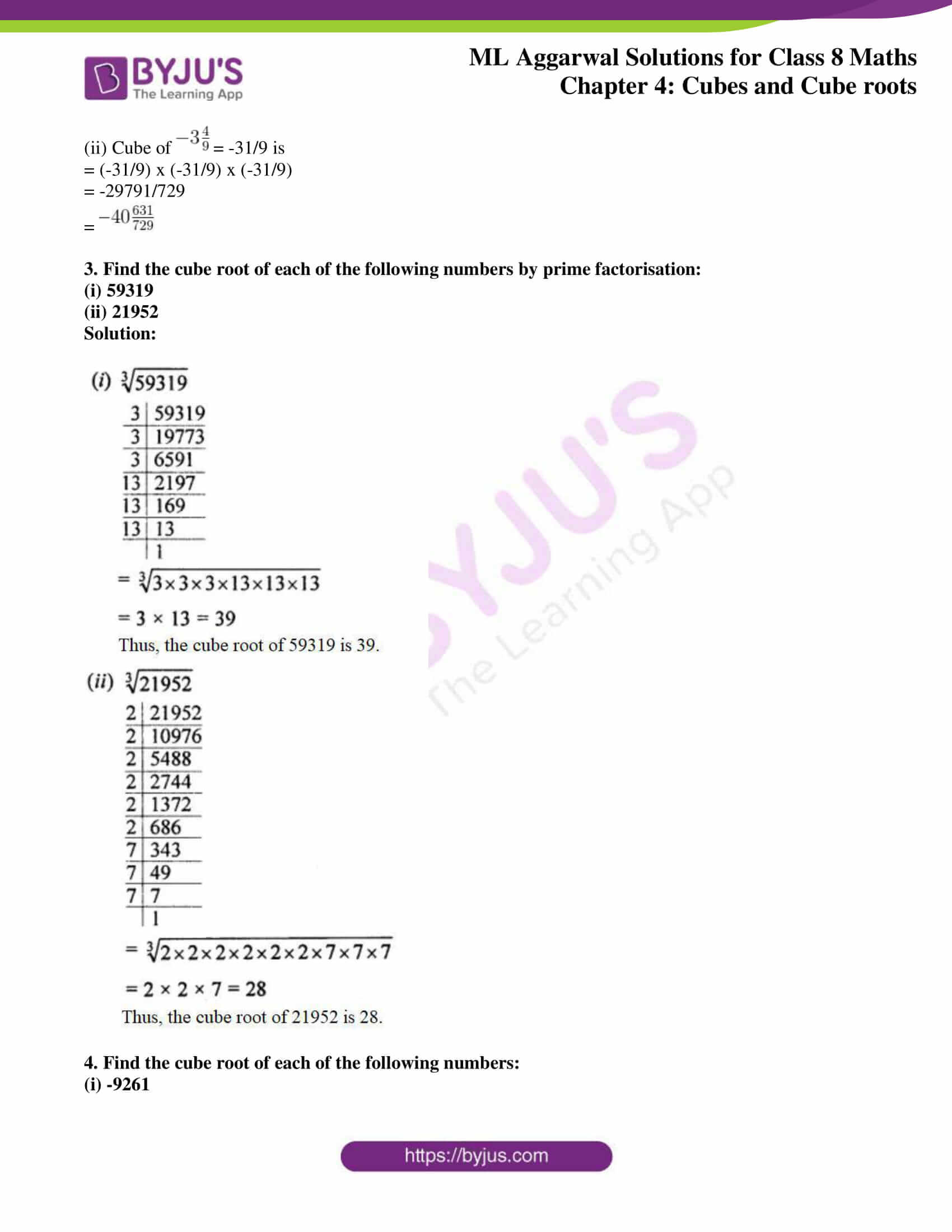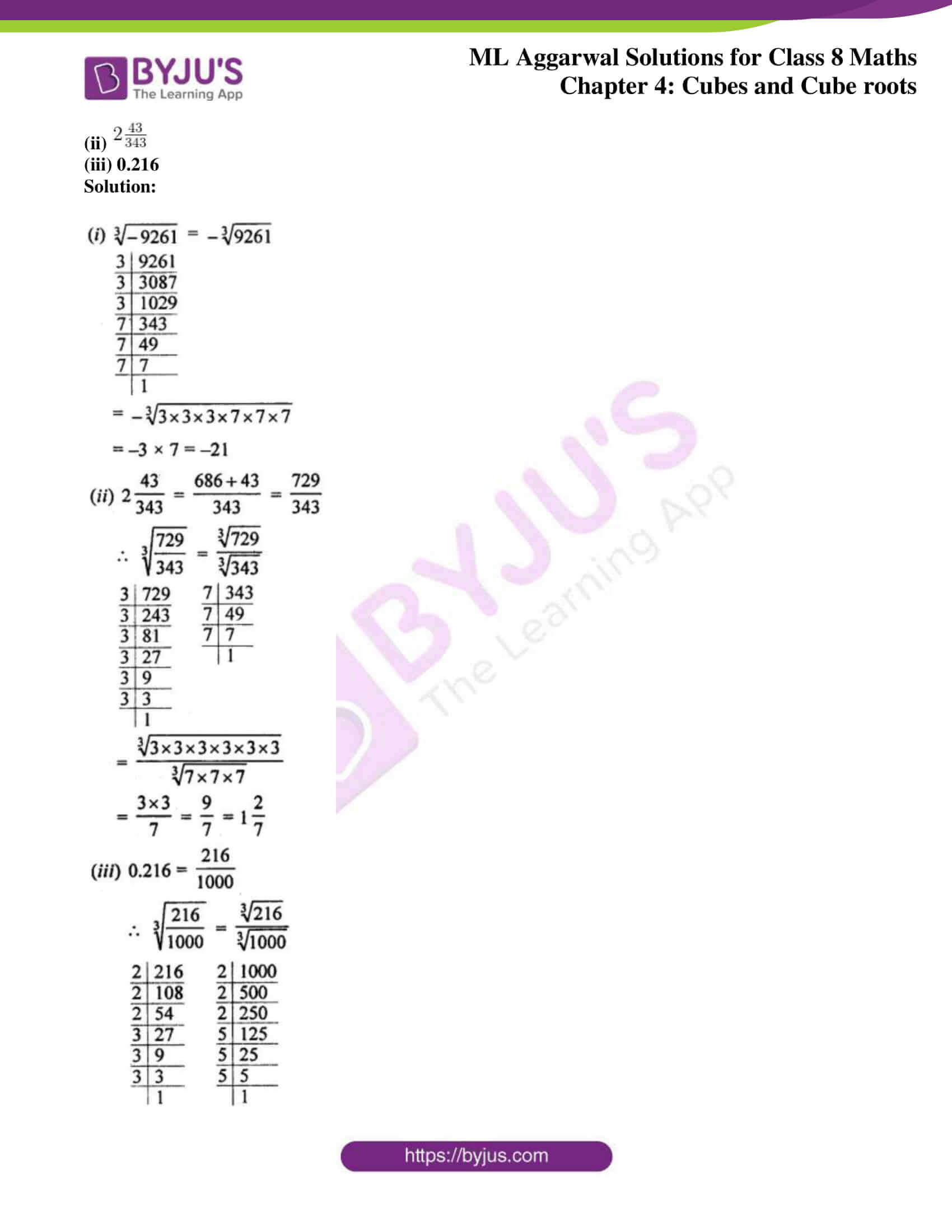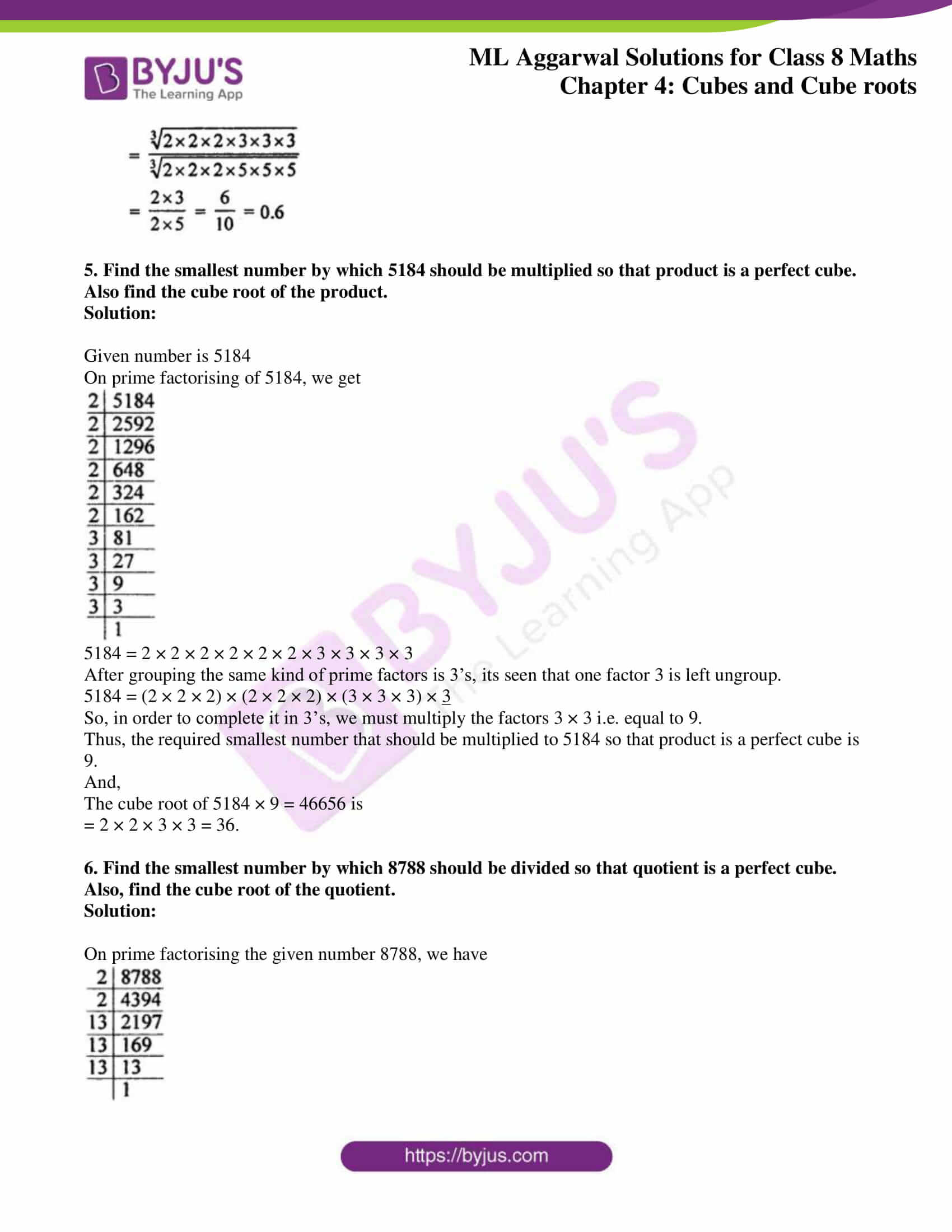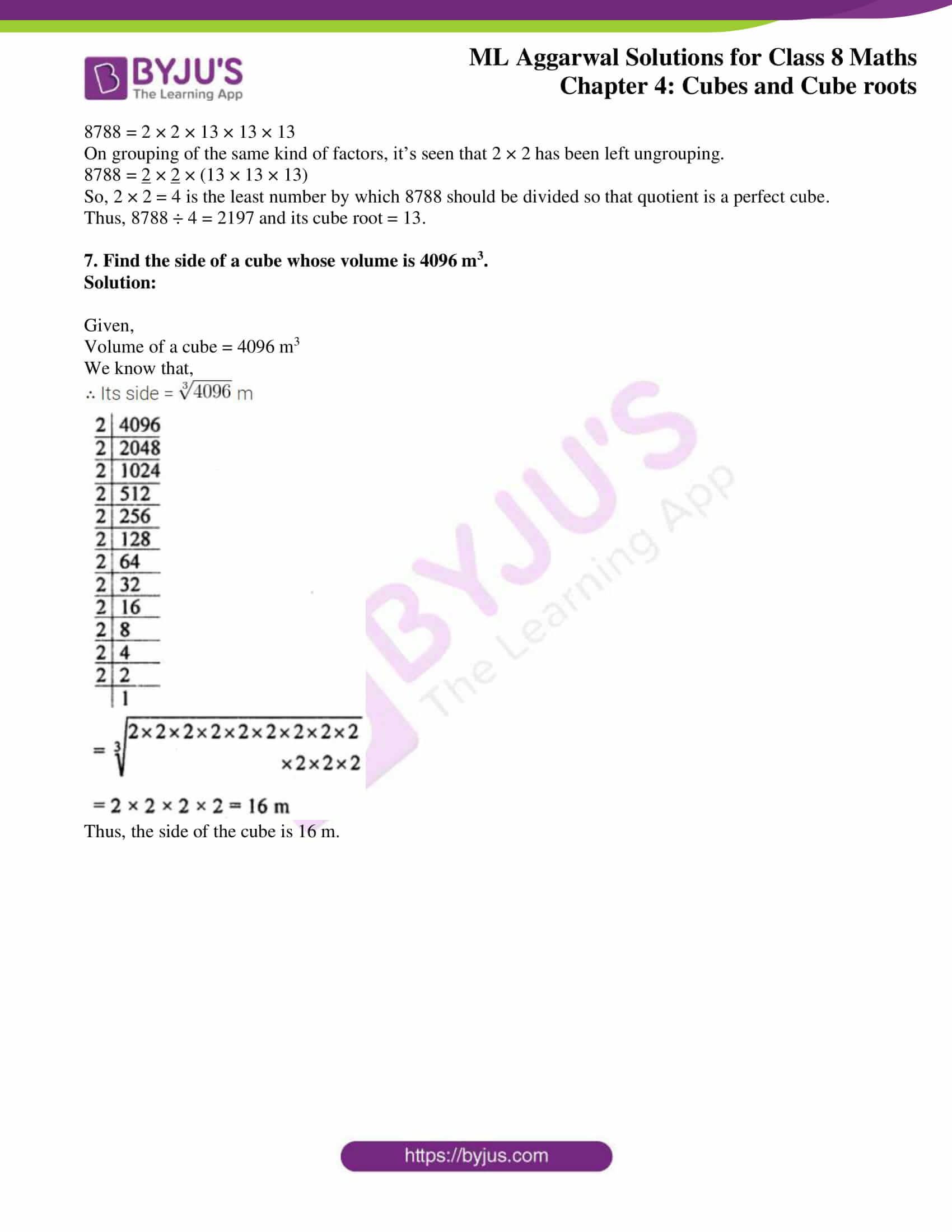### Access answers to ML Aggarwal Solutions for Class 8 Maths Chapter 4 Cubes and Cube Roots

Exercise 4.1

1. Which of the following numbers are not perfect cubes? Give reasons in support of your answer:
(i) 648
(ii) 729
(iii) 8640
(iv) 8000
Solution:

(i) We have,

648 = 2 × 2 × 2 × 3 × 3 × 3 × 3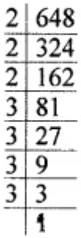After grouping the prime factors in triplets, one factor 3 is left without grouping.

648 = (2 × 2 × 2) × (3 × 3 × 3) × 3

Thus, 648 is not a perfect cube.

(ii) We have,

729 = 3 × 3 × 3 × 3 × 3 × 3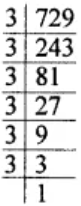After grouping the prime factors in triplets, it’s seen that no factor is left.

729 = (3 × 3 × 3) × (3 × 3 × 3)

Thus, 729 is a perfect cube.

(iii) We have,

8640 = 2 × 2 × 2 × 2 × 2 × 2 × 3 × 3 × 3 × 5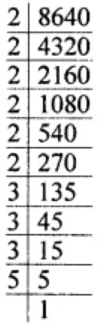After grouping the prime factors in triplets, it’s seen that one factor 5 is left without grouping.

8640 = (2 × 2 × 2) × (2 × 2 × 2) × (3 × 3 × 3) × 5

Thus, 8640 is not a perfect cube.

(iv) We have,

8000 = 2 × 2 × 2 × 2 × 2 × 2 × 5 × 5 × 5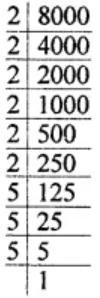After grouping the prime factors in triplets, it’s seen that no factor is left.

8000 = (2 × 2 × 2) × (2 × 2 × 2) × (5 × 5 × 5)

Thus, 8000 is a perfect cube.

2. Show that each of the following numbers is a perfect cube. Also, find the number whose cube is the given number:
(i) 1728
(ii) 5832
(iii) 13824
(iv) 35937
Solution:

(i) We have,

1728 = 2 × 2 × 2 × 2 × 2 × 2 × 3 × 3 × 3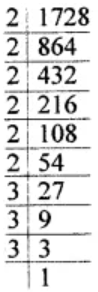After grouping the prime factors in triplets, it’s seen that no factor is left without grouping.

1728 = (2 × 2 × 2) × (2 × 2 × 2) × (3 × 3 × 3)

Thus, 1728 is a perfect cube and its cube root is 2 × 2 × 3 = 12.

(ii) We have,

5832 = 2 × 2 × 2 × 3 × 3 × 3 × 3 × 3 × 3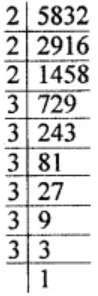After grouping the prime factors in triplets, it’s seen that no factor is left without grouping.

5832 = (2 × 2 × 2) × (3 × 3 × 3) × (3 × 3 × 3)

Thus, 5832 is a perfect cube and its cube root is 2 × 3 × 3 = 18

(iii) We have,

13824 = 2 × 2 × 2 × 2 × 2 × 2 × 2 × 2 × 2 × 3 × 3 × 3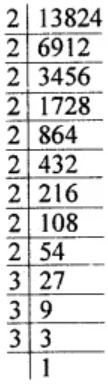After grouping the prime factors in triplets, its seen that no factor is left without grouping.

13824 = (2 × 2 × 2) × (2 × 2 × 2) × (2 × 2 × 2) × (3 × 3 × 3)

Thus, 13824 is a perfect cube and its cube root is 2 × 2 × 2 × 3 = 24.

(iv) We have,

35937 = 3 × 3 × 3 × 11 × 11 × 11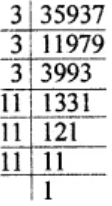After grouping the prime factors in triplets, it’s seen that no factor is left without grouping.

35937 = (3 × 3 × 3) × (11 × 11 × 11)

Thus, 35937 is a perfect cube and its cube root is 3 × 11 = 33

3. Find the smallest number by which each of the following numbers must be multiplied to obtain a perfect cube:
(i) 243
(ii) 3072
(iii) 11979
(iv) 19652
Solution:

(i) We have,

243 = 3 × 3 × 3 × 3 × 3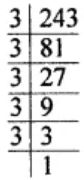After grouping the prime factors in triplets, it’s seen that factors 3 × 3 are left.

243 = (3 × 3 × 3) × 3 × 3

So, in order to complete in a group of 3’s, one more factor of 3 is needed.

Thus, the smallest number which should be multiplied to 243 in order to make it a perfect cube is 3.

(ii) We have,

3072 = 2 × 2 × 2 × 2 × 2 × 2 × 2 × 2 × 2 × 2 × 3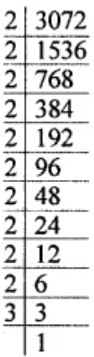After grouping the prime factors in triplets, it’s seen that factor 2 × 3 are left ungrouped.

3072 = (2 × 2 × 2) × (2 × 2 × 2) × (2 × 2 × 2) × 2 × 3

So, in order to complete them in a group of 3’s we need the factors 2 × 2 × 3 × 3 to be multiplied.

i.e. the factor needed is 2 × 2 × 3 × 3 = 36

Thus, the smallest number which should be multiplied to 3072 in order to make it a perfect cube is 36.

(iii) We have,

11979 = 3 × 3 × 11 × 11 × 11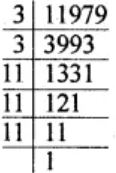After grouping the prime factors in triplets, it’s seen that factors 3 × 3 are left without grouping in 3’s.

11979 = 3 × 3 × (11 × 11 × 11)

So, in order to complete in a group of 3’s, one more factor of 3 is needed.

Thus, the smallest number which should be multiplied to 11979 in order to make it a perfect cube is 3.

(iv) We have,

19652 = 2 × 2 × 17 × 17 × 17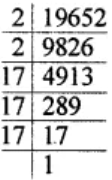After grouping the prime factors in triplets, it’s seen that factors 2 × 2 are left ungrouped in 3’s.

19652 = 2 × 2 × (17 × 17 × 17)

So, in order to complete it in a triplet one more 2 is needed.

Thus, the smallest number which should be multiplied to 19652 in order to make it a perfect cube is 2.

4. Find the smallest number by which each of the following numbers must be divided to obtain a perfect cube:
(i) 1536
(ii) 10985
(iii) 28672
(iv) 13718
Solution:

(i) We have,

1536 = 2 × 2 × 2 × 2 × 2 × 2 × 2 × 2 × 2 × 3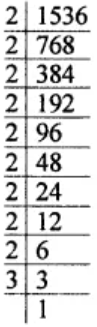After grouping the prime factors in triplets, it’s seen that one factor 3 is left without grouping.

1536 = (2 × 2 × 2) × (2 × 2 × 2) × (2 × 2 × 2) × 3

So, in order to make it a perfect cube, it must be divided by 3.

Thus, the smallest number by which 1536 must be divided to obtain a perfect cube is 3.

(ii) We have,

10985 = 5 × 13 × 13 × 13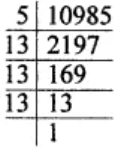After grouping the prime factors in triplet, it’s seen that one factor 5 is left without grouping.

10985 = 5 × (13 × 13 × 13)

So, it must be divided by 5 in order to get a perfect cube.

Thus, the required smallest number is 5.

(iii) We have,

28672 = 2 × 2 × 2 × 2 × 2 × 2 × 2 × 2 × 2 × 2 × 2 × 2 × 7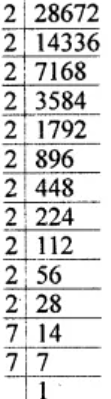After grouping the prime factors in triplets, it’s seen that one factor 7 is left without grouping.

28672 = (2 × 2 × 2) × (2 × 2 × 2) × (2 × 2 × 2) × (2 × 2 × 2) × 7

So, it must be divided by 7 in order to get a perfect cube.

Thus, the required smallest number is 7.

(iv) 13718 = 2 × 19 × 19 × 19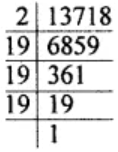After grouping the prime factors in triplets, it’s seen that one factor 2 is left without grouping.

13718 = 2 × (19 × 19 × 19)

So, it must be divided by 2 in order to get a perfect cube.

Thus, the required smallest number is 2.

5. Rahul makes a cuboid of plasticine of sides 3 cm × 3 cm × 5 cm. How many such cuboids will he need to form a cube?
Solution:

Given,

Cuboid with dimensions 3 cm × 3 cm × 5 cm

To form into a cube, number of such cuboid required are

= (15/3) x (15/3) x (15/5)

= 5 × 5 × 3

= 75

Thus, the number of cuboids required to make a cube is 75.

6. Find the volume of a cubical box whose surface area is 486 cm2.
Solution:

Given,

Surface area of a cubical box = 486 cm2

We know that,

Surface area of a cubical box = 6 x (side)2

So,

Side = √(486/6) = 9 cm

Now, volume = (Side)3

= (9)3

= 9 × 9 × 9 = 729 cm3

Thus, the volume of the cubical box is 729 cm3.

7. Which of the following are cubes of even natural numbers or odd natural numbers:
(i) 125
(ii) 512
(iii) 1000
(iv) 2197
(v) 4096
(vi) 6859
Solution:

We know that,

The cube of an even number is even and the cube of an odd number is odd.

Hence,

125, 2197, 6859 are cubes of an odd number and 512, 1000, 4096 are cubes of an even number.

8. Write the ones digit of the cube of each of the following numbers:
(i) 231
(ii) 358
(iii) 419
(iv) 725
(v) 854
(vi) 987
(vii) 752
(viii) 893
Solution:

We know that,

The cube of number having 1, 4, 5, 6 or 9 in unit place will end in 1, 4, 5, 6 or 9

And, if the numbers have:

2 in unit place, then it’s cube ends in 8

8 in unit place, then it’s cube ends in 2

3 in unit place then it’s cube ends in 7

7 in unit place then it’s cube ends in 3

0 in unit place then it’s cube ends in 0.

So now,

(i) Unit digit of number 231 is 1, hence its cube will end in 1.

(ii) Unit digit of number 358 is 8, hence its cube will end in 2.

(iii) Unit digit of number 419 is 9, hence its cube will end in 9.

(iv) Unit digit of number 725 is 5, hence its cube will end in 5.

(v) Unit digit of number 854 is 4, hence its cube will end in 4.

(vi) Unit digit of number 987 is 7, hence its cube will end in 3.

(vii) Unit digit of number 752 is 2, hence its cube will end in 8.

(viii) Unit digit of numbers 893 is 3, hence its cube will end in 7.

9. Find the cubes of the following numbers:
(i) -13
(ii)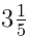(iii)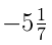Solution:

(i) Cube of -13 = (-13)3

= (-13) × (-13) × (-13)

= -2197

(ii) Cube of= (16/5)3 = (16 x 16 x 16)/ (5 x 5 x 5)

= 4096/125

=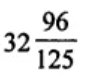(iii) Cube of= (-36/7)3 = (-36 x -36 x -36)/ (7 x 7 x 7)

= -46656/49

=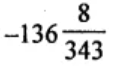Exercise 4.2

1. Find the cube root of each of the following numbers by prime factorization:
(i) 12167
(ii) 35937
(iii) 42875
(iv) 21952
(v) 373248
(vi) 32768
(vii) 262144
(viii) 157464
Solution: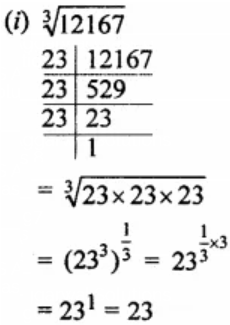Thus, the cube root of 12167 is 23.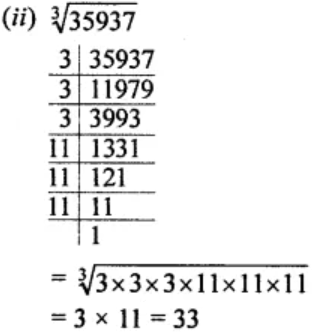Thus, the cube root of 35937 is 33.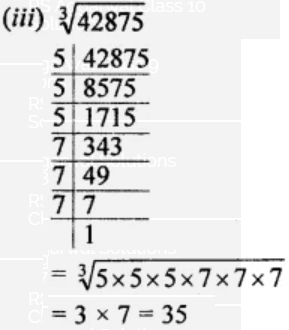Thus, the cube root of 42875 is 35.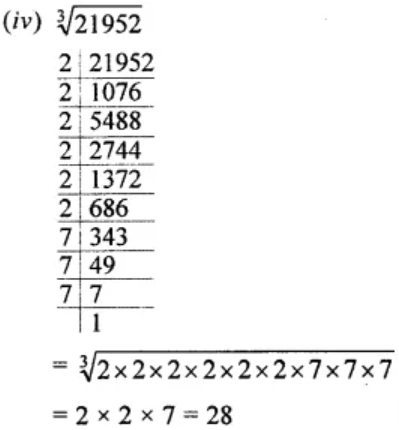Thus, the cube root of 21952 is 28.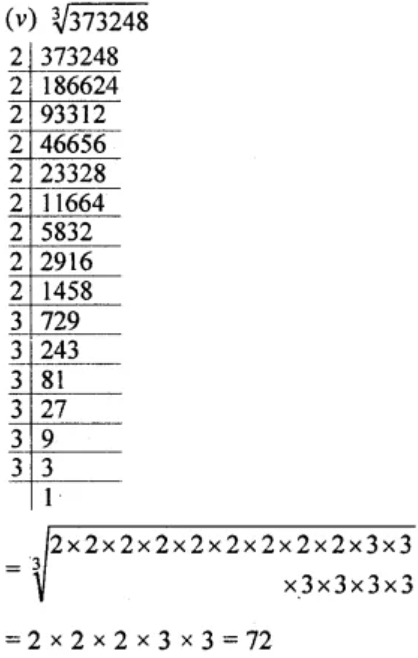Thus, the cube root of 373248 is 72.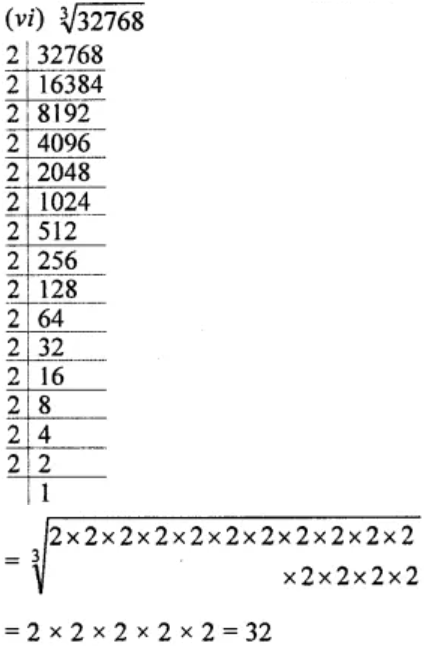Thus, the cube root of 32768 is 32.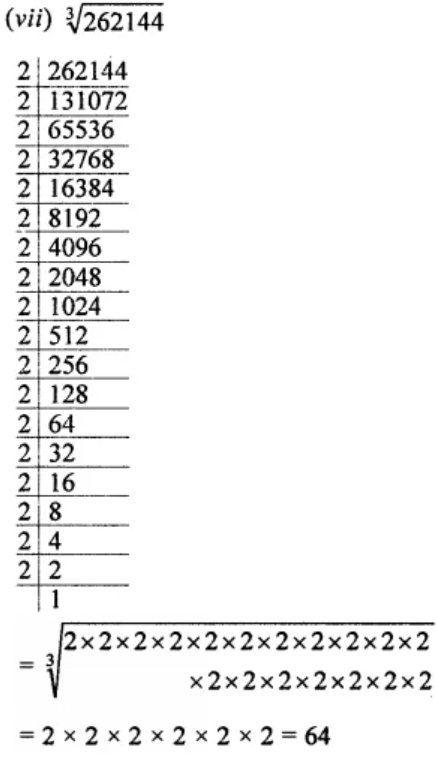Thus, the cube root of 262144 is 64.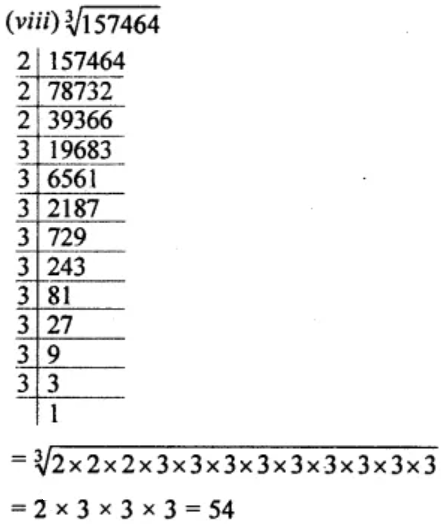Thus, the cube root of 157464 is 54.

2. Find the cube root of each of the following cube numbers through estimation.
(i) 19683
(ii) 59319
(iii) 85184
(iv) 148877
Solution:

(i) 19683

Grouping in 3’s from right to left, we have 19,683

In the first group, 683 the unit digit is 3

So, the cube root will end with 7

And in the second group, 19

Cubing 23 = 8 and 33 = 27

As, 8 < 19 < 27

The ten’s digit of the cube root will be 2

Thus, the cube root of 19683 is 27.

(ii) 59319

Grouping in 3’s from right to left, we have 59,319

In first group 319, unit digit is 9

So, the unit digit of its cube root will be 9

And in the second group, 59

Cubing 33 = 27 and 43 = 64

As, 27 < 59 < 64

The ten’s digit of the cube root will be 3

Thus, the cube root of 59319 is 39.

(iii) 85184

Grouping in 3’s from right to left, we have 85,184

In the first group 184, the unit digit is 4

So, the unit digit of its cube root will be 4

And in the second group, 85

Cubing 43 = 64 and 53 = 125

As, 64 < 85 < 125

The ten’s digit of cube root will be 4

Thus, the cube root of 85184 is 44.

(iv) 148877

Grouping in 3’s, from right to left, we have 148,877

In the first group 877, unit digit is 7

So, the unit digit of cube root will be 3

And in the second group, 148

Cubing 53 = 125, 63 = 216

125 < 148 < 216

The ten’s digit of cube root will be 5

Thus, the cube root of 148877 is 53.

3. Find the cube root of each of the following numbers:

(i) -250047 (ii) -64/1331

(iii)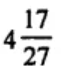(iv)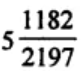Solution:Thus, the cube root of -250047 is -63.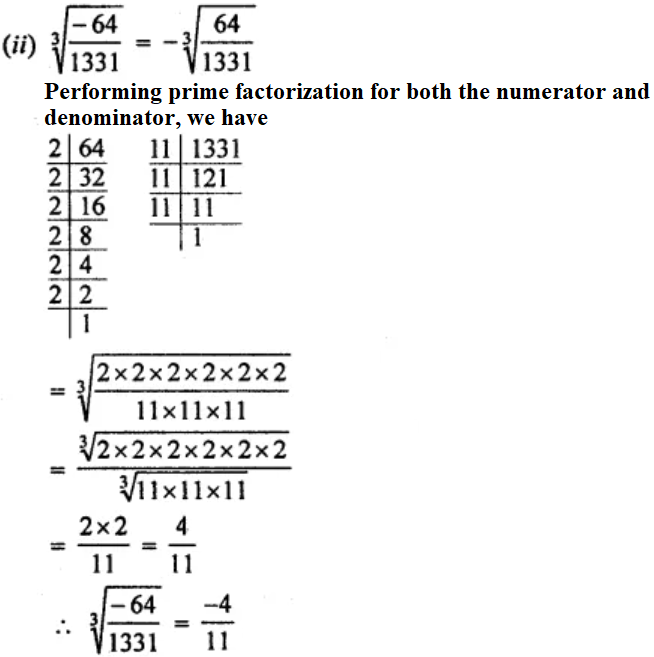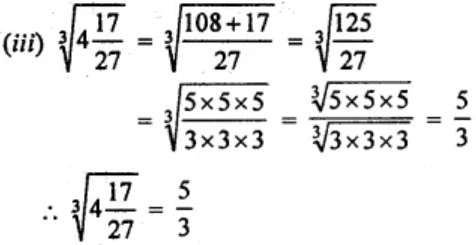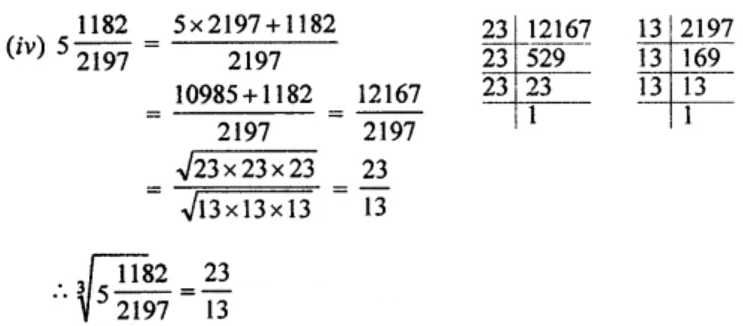4. Evaluate the following: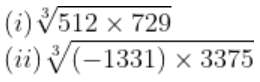Solution: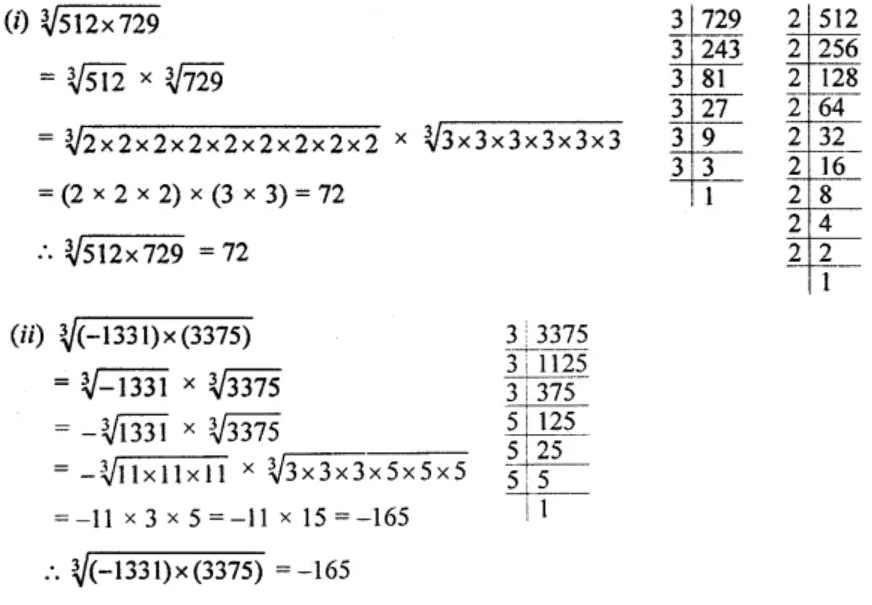5. Find the cube root of the following decimal numbers:
(i) 0.003375
(ii) 19.683
Solution: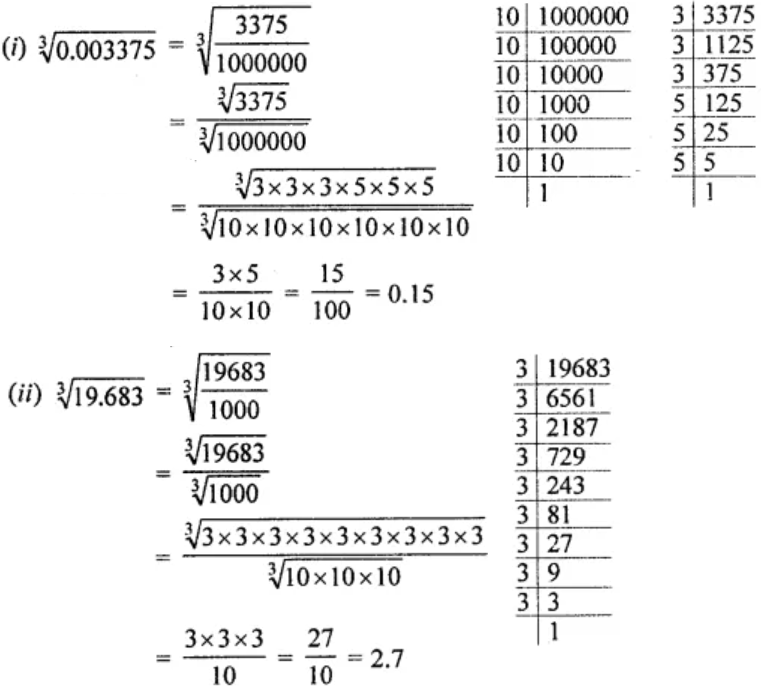6.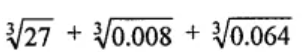Solution: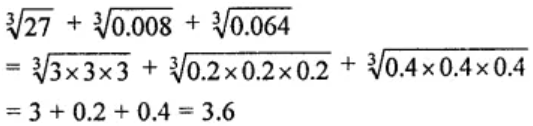7. Multiply 6561 by the smallest number so that product is a perfect cube. Also, find the cube root of the product.
Solution:

Performing prime factorization of 6561, we get

6561 = 3 × 3 × 3 × 3 × 3 × 3 × 3 × 3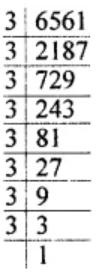6561 = (3 × 3 × 3) × (3 × 3 × 3) × 3 × 3

After grouping of the equal factors in 3’s, it’s seen that 3 × 3 is left ungrouped in 3’s.

In order to complete it in triplet, we should multiply it by 3.

Hence, required smallest number = 3

and cube root of the product = 3 × 3 × 3 = 27

8. Divide the number 8748 by the smallest number so that the quotient is a perfect cube. Also, find the cube root of the quotient.
Solution:

Given number is 8748

On prime factorising, we get

8748 = 2 × 2 × 3 × 3 × 3 × 3 × 3 × 3 × 3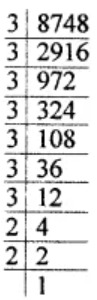Grouping of the equal factor in 3’s, it’s seen that 2 × 2 × 3 is left without grouping.

8748 = 2 × 2 × 3 × (3 × 3 × 3) × (3 × 3 × 3)

Hence, on dividing the number 8748 by 12, we get 729

And, the cube root of 729 is 3 × 3 = 9.

9. The volume of a cubical box is 21952 m3. Find the length of the side of the box.
Solution:

Given, the volume of a cubical box is 21952 m2.

We know that,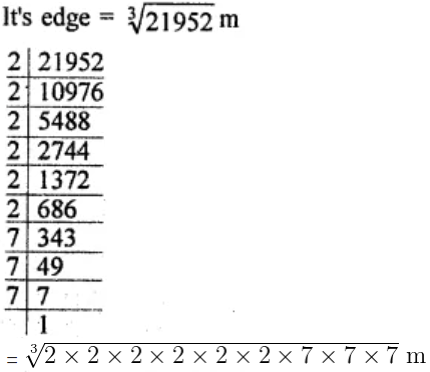= 2 × 2 × 7 = 28 m

Thus, the length of the side of the box is 28 m.

10. Three numbers are in the ratio 3 : 4 : 5. If their product is 480, find the numbers.
Solution:

Given,

Three numbers are in the ratio 3:4:5 and their product = 480

Let’s assume the numbers to be 3x, 4x and 5x, then we have

3x × 4x × 5x = 480

⇒ 60 x3 = 480

⇒ x3 = 480/60 = 8 = (2)3

∴ x = 2

Thus, the number are 2 × 3, 2 × 4 and 2 × 5 = 6, 8 and 10

11. Two numbers are in the ratio 4 : 5. If difference of their cubes is 61, find the numbers.
Solution:

Given,

Two numbers are in the ratio = 4 : 5

Difference between their cubes = 61

Let’s assume the numbers to be 4x and 5x

So, we have

(5x)3 – (4x)3 = 61

125x3 – 64x3 = 61

61x3 = 61

⇒ x3 = 1 = (1)3

∴ x = 1

Hence, 4x = 4 × 1 = 4 and 5x = 5 × 1 = 5

Therefore, the numbers are 4 and 5

12. Difference of two perfect cubes is 387. If the cube root of the greater of two numbers is 8, find the cube root of the smaller number.
Solution:

Given,

The difference in two cubes = 387

And, the cube root of the greater number = 8

So, the greater number = (8)3 = 8 × 8 × 8 = 512

Hence, the second number = 512 – 387 = 125

Thus,

The cube root of 125 is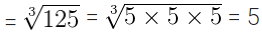1. Show that each of the following numbers is a perfect cube. Also find the number whose cube is the given number:
(i) 74088
(ii) 15625
Solution:

(i) The prime factorization of 74088 is given by,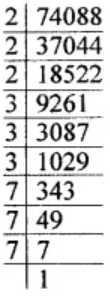= 2 × 2 × 2 × 3 × 3 × 3 × 7 × 7 × 7

And, after grouping the same kind of prime factors in 3’s, its seen that no factor has been left ungrouped.

74088 = (2 × 2 × 2) × (3 × 3 × 3) × (7 × 7 × 7)

Thus, 74088 is a perfect cube and its cube root is 2 × 3 × 7 = 42.

(ii) The prime factorization of 15625 is given by,= 5 × 5 × 5 × 5 × 5 × 5

And, after grouping the same kind of prime factors, its seen that no factor is left ungrouped.

15625 = (5 × 5 × 5) × (5 × 5 × 5)

Thus, 15625 is a perfect cube and its cube root is 5 × 5 = 25.

2. Find the cube of the following numbers:
(i) -17
(ii)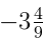Solution:

(i) Cube of -17 = (-17) × (-17) × (-17)

= -4913

(ii) Cube of= -31/9 is

= (-31/9) x (-31/9) x (-31/9)

= -29791/729

=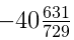3. Find the cube root of each of the following numbers by prime factorisation:
(i) 59319
(ii) 21952
Solution: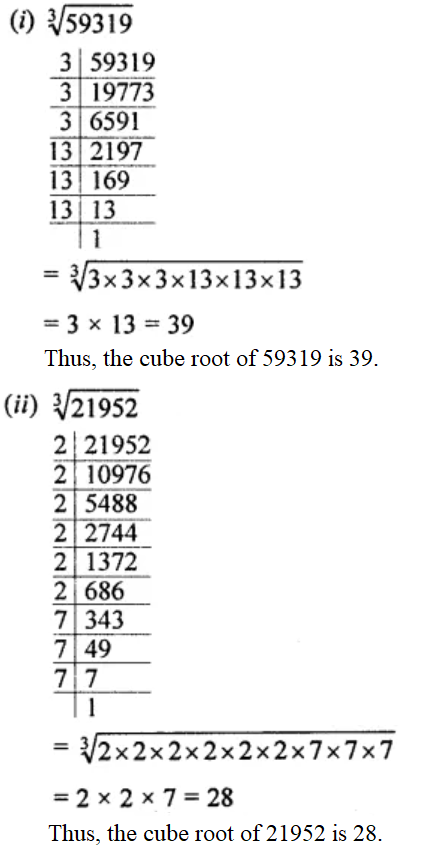4. Find the cube root of each of the following numbers:
(i) -9261
(ii)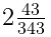(iii) 0.216
Solution: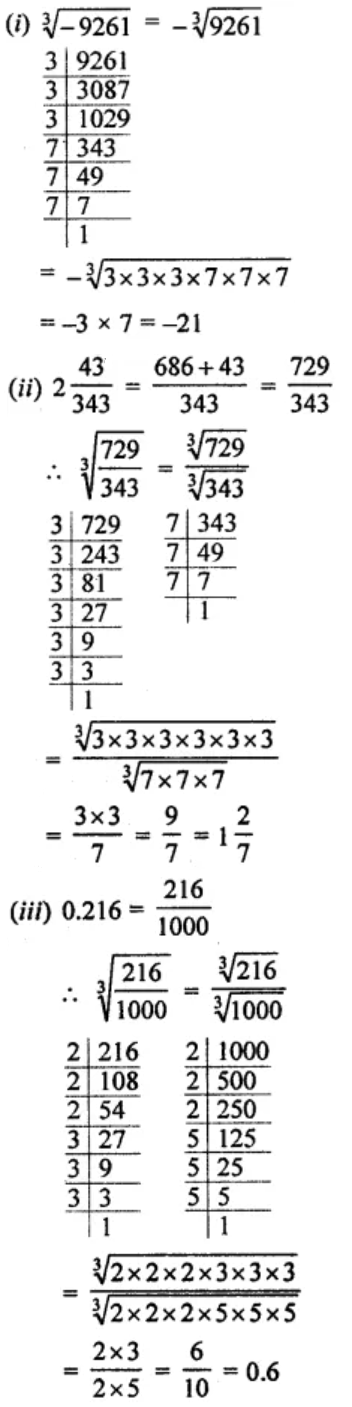5. Find the smallest number by which 5184 should be multiplied so that product is a perfect cube. Also find the cube root of the product.
Solution:

Given number is 5184

On prime factorising of 5184, we get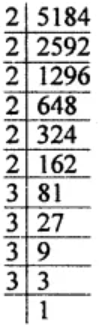5184 = 2 × 2 × 2 × 2 × 2 × 2 × 3 × 3 × 3 × 3

After grouping the same kind of prime factors is 3’s, its seen that one factor 3 is left ungroup.

5184 = (2 × 2 × 2) × (2 × 2 × 2) × (3 × 3 × 3) × 3

So, in order to complete it in 3’s, we must multiply the factors 3 × 3 i.e. equal to 9.

Thus, the required smallest number that should be multiplied to 5184 so that product is a perfect cube is 9.

And,

The cube root of 5184 × 9 = 46656 is

= 2 × 2 × 3 × 3 = 36.

6. Find the smallest number by which 8788 should be divided so that quotient is a perfect cube. Also, find the cube root of the quotient.
Solution:

On prime factorising the given number 8788, we have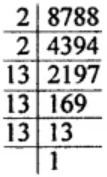8788 = 2 × 2 × 13 × 13 × 13

On grouping of the same kind of factors, it’s seen that 2 × 2 has been left ungrouping.

8788 = 2 × 2 × (13 × 13 × 13)

So, 2 × 2 = 4 is the least number by which 8788 should be divided so that quotient is a perfect cube.

Thus, 8788 ÷ 4 = 2197 and its cube root = 13.

7. Find the side of a cube whose volume is 4096 m3.
Solution:

Given,

Volume of a cube = 4096 m3

We know that,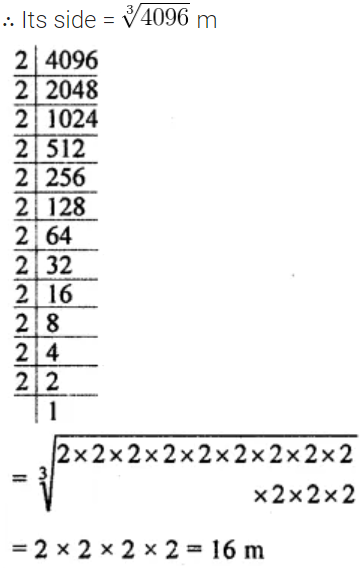Thus, the side of the cube is 16 m.